# Lakhmir Singh Solutions Class 10 Chemistry Chapter 5 Periodic Classification Of Elements

There are 115 elements known at present. The elements are classified into different periods and groups, according to their properties. It was very difficult in an earlier period of time for scientists to study the properties of elements separately and dividing them into groups, based on their nature. Dobereiner’s triads were the earlier attempt to classify the elements based on their unique property, but it had many drawbacks. Then, Newland put forth the law of arranging the known elements in the order of increasing mass known as Newlands law of octaves.

Later, Mendeleev came up with an acceptable concept and made a periodic law.

This chapter is all about the arrangement of elements according to their characteristic properties, merits and demerits of various concepts of classification and the modern periodic table.

## Download PDF Of Lakhmir Singh Solutions for Class 10 Chemistry Chapter 5 Periodic Classification Of Elements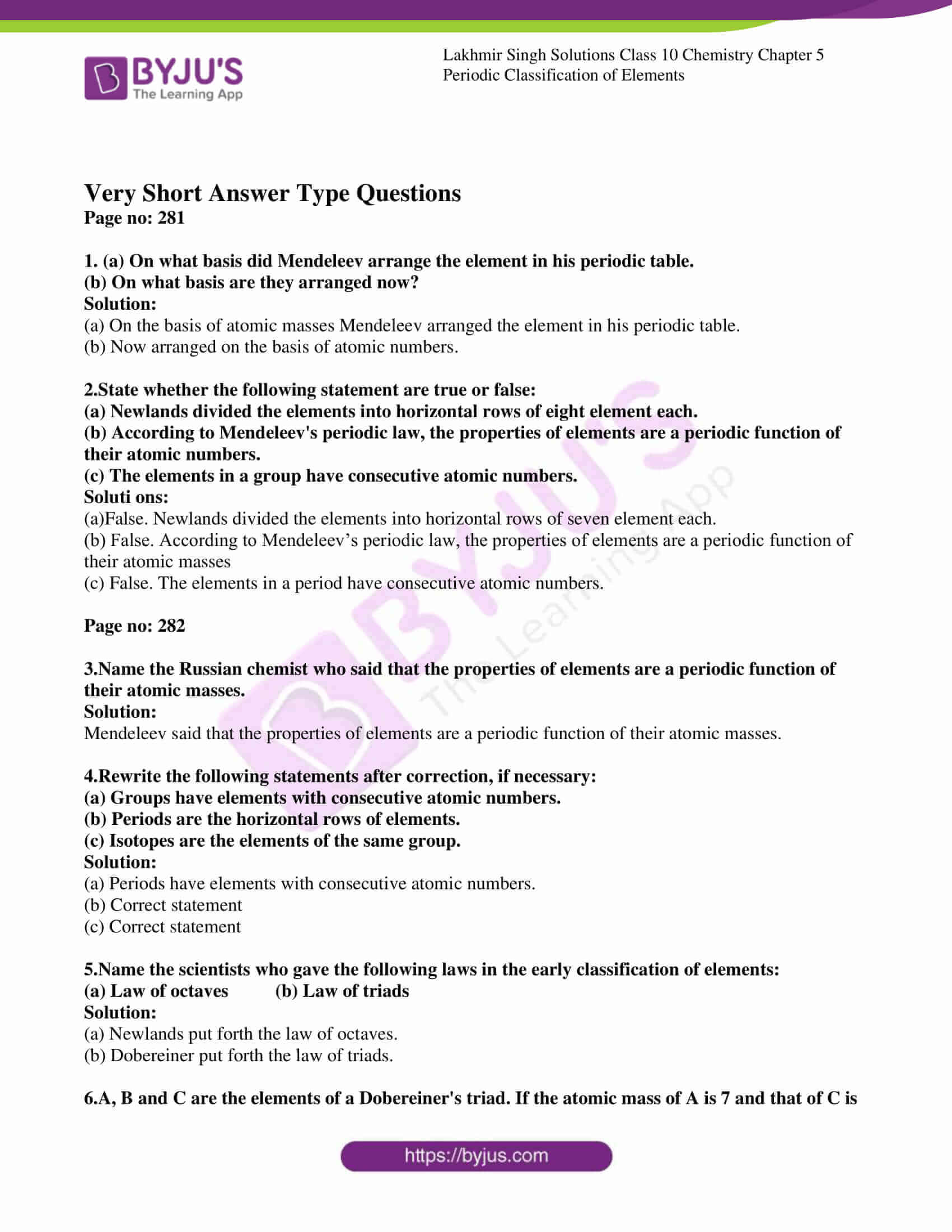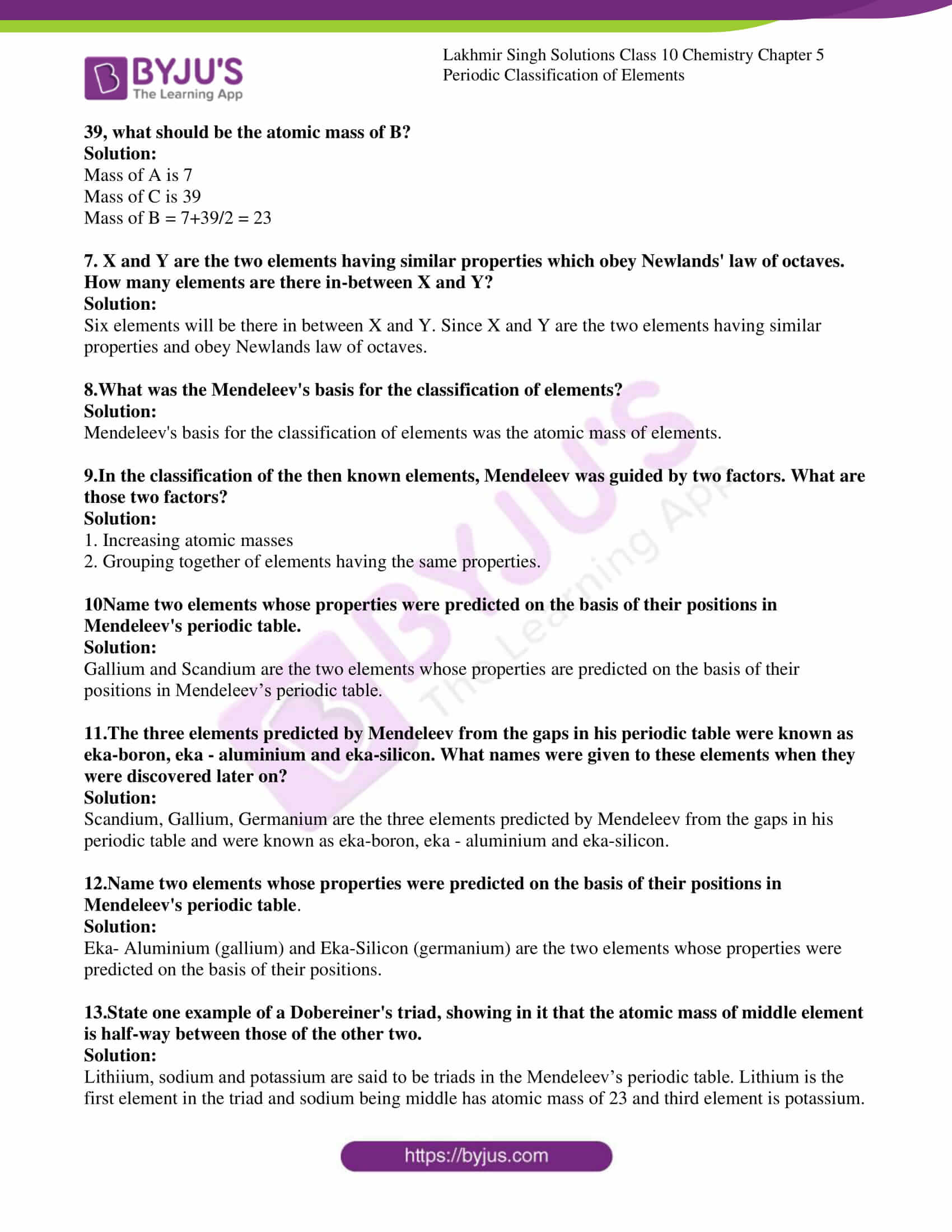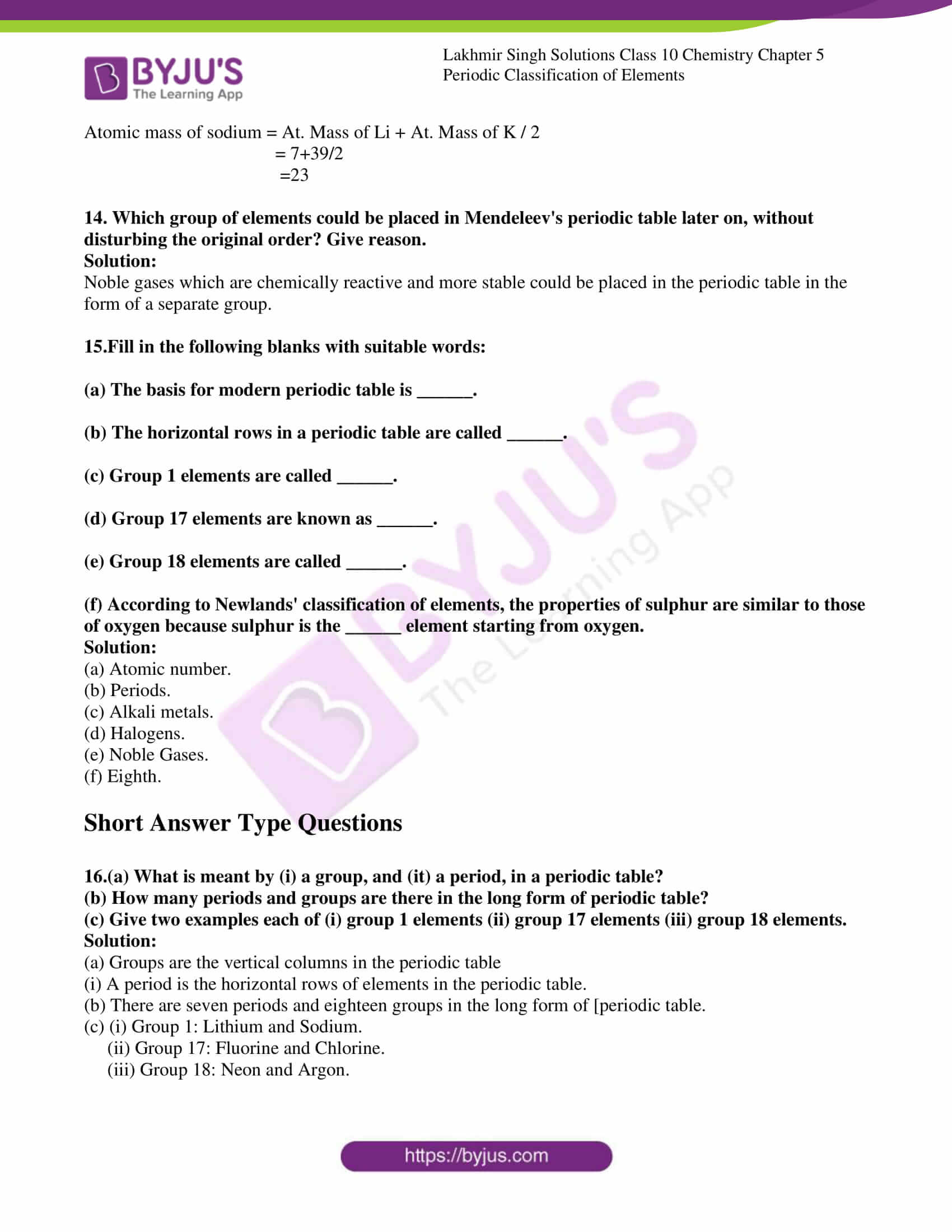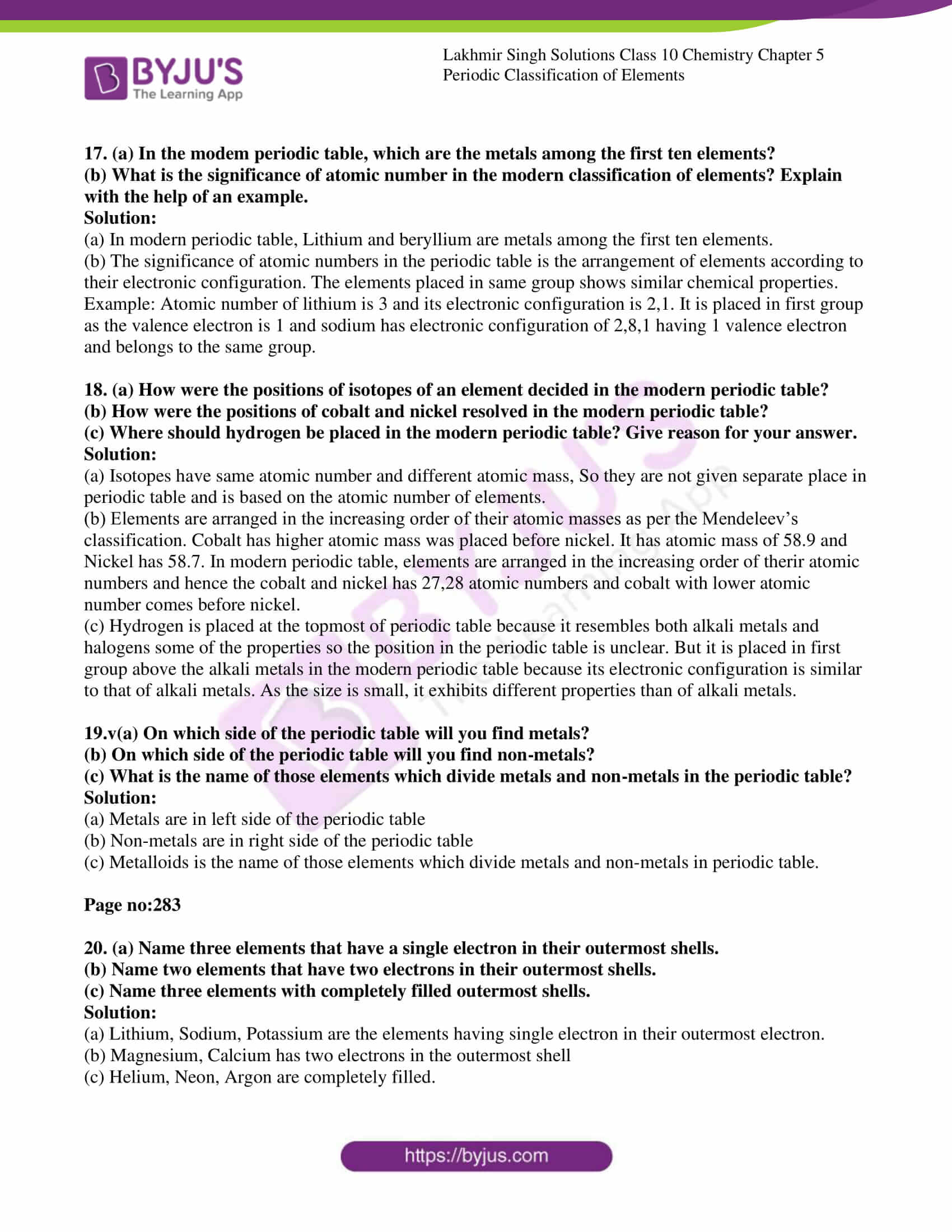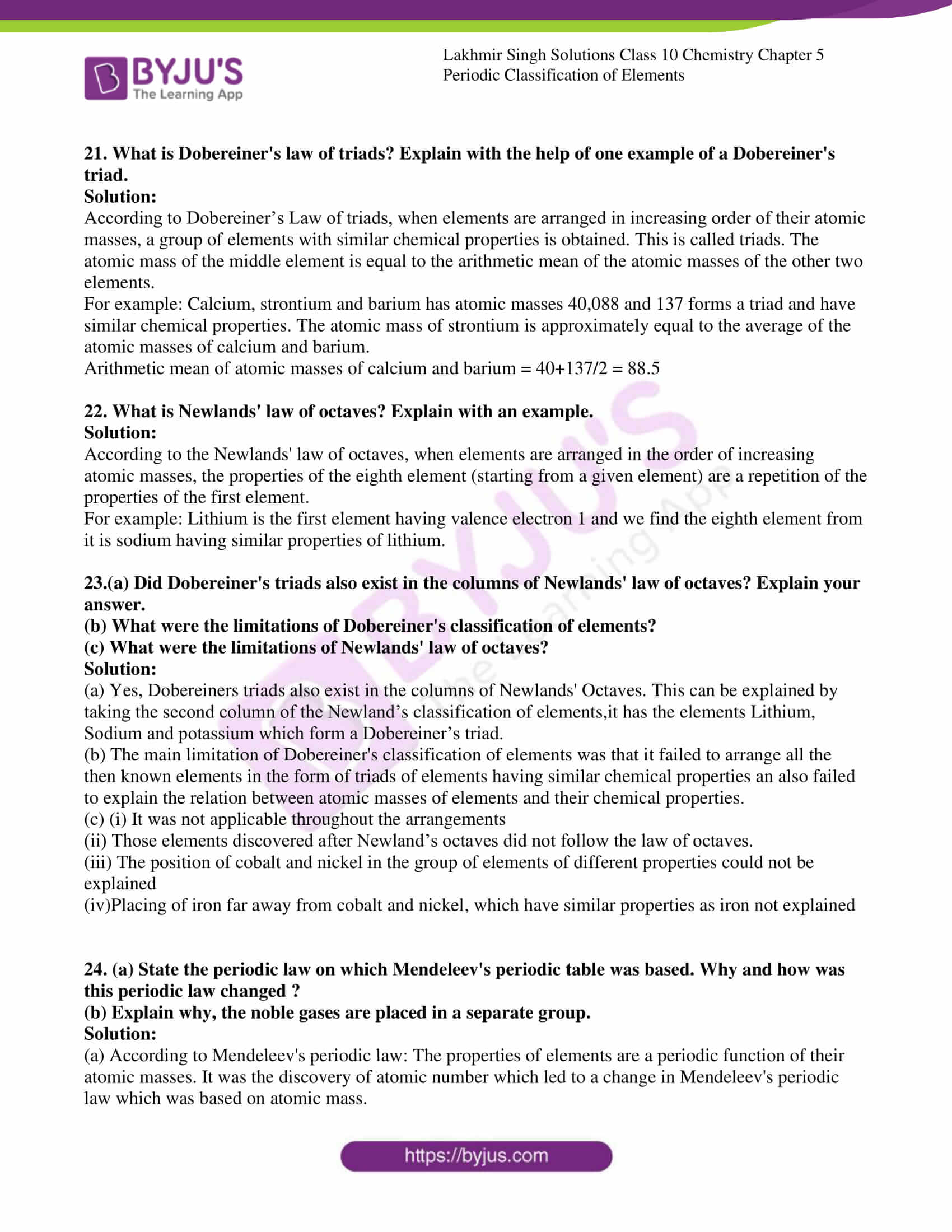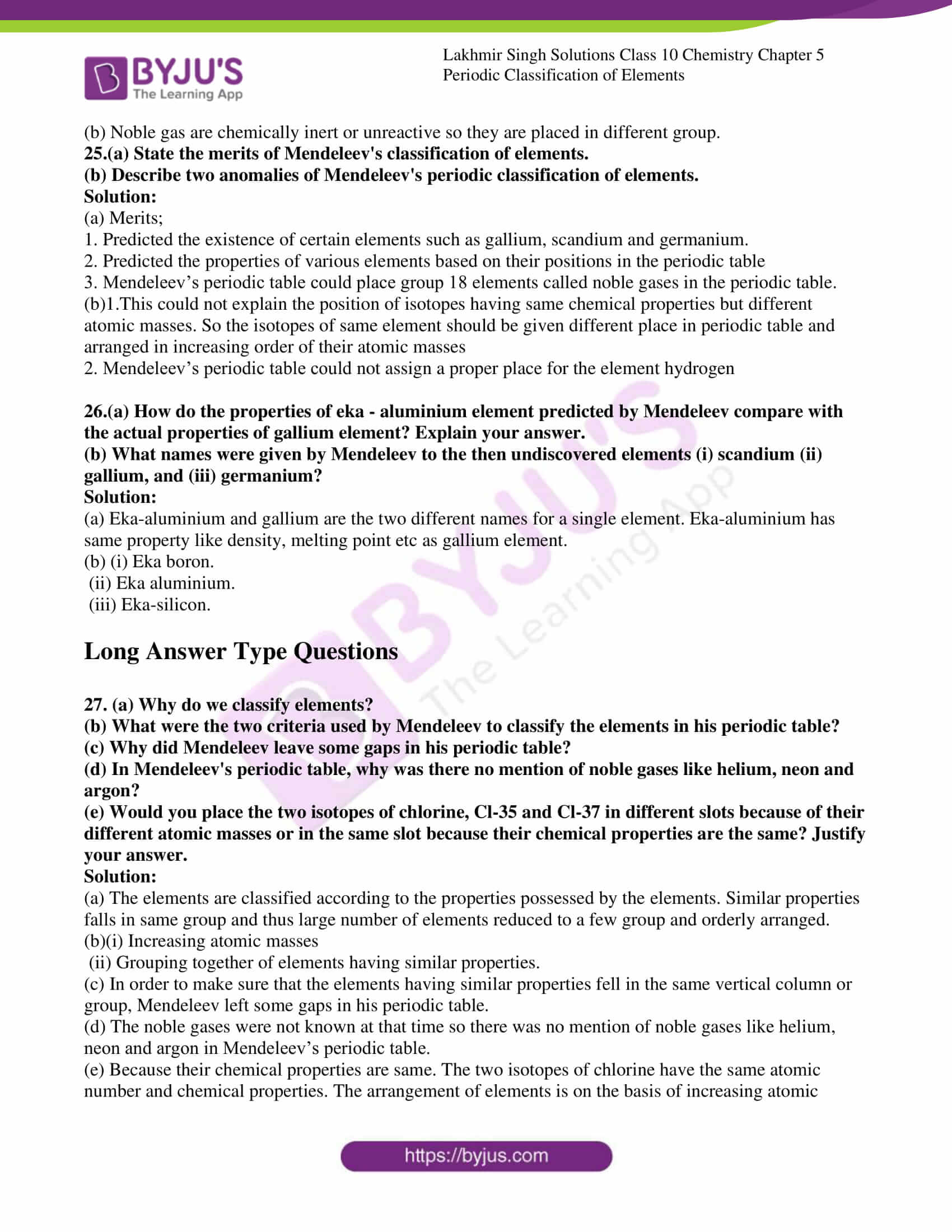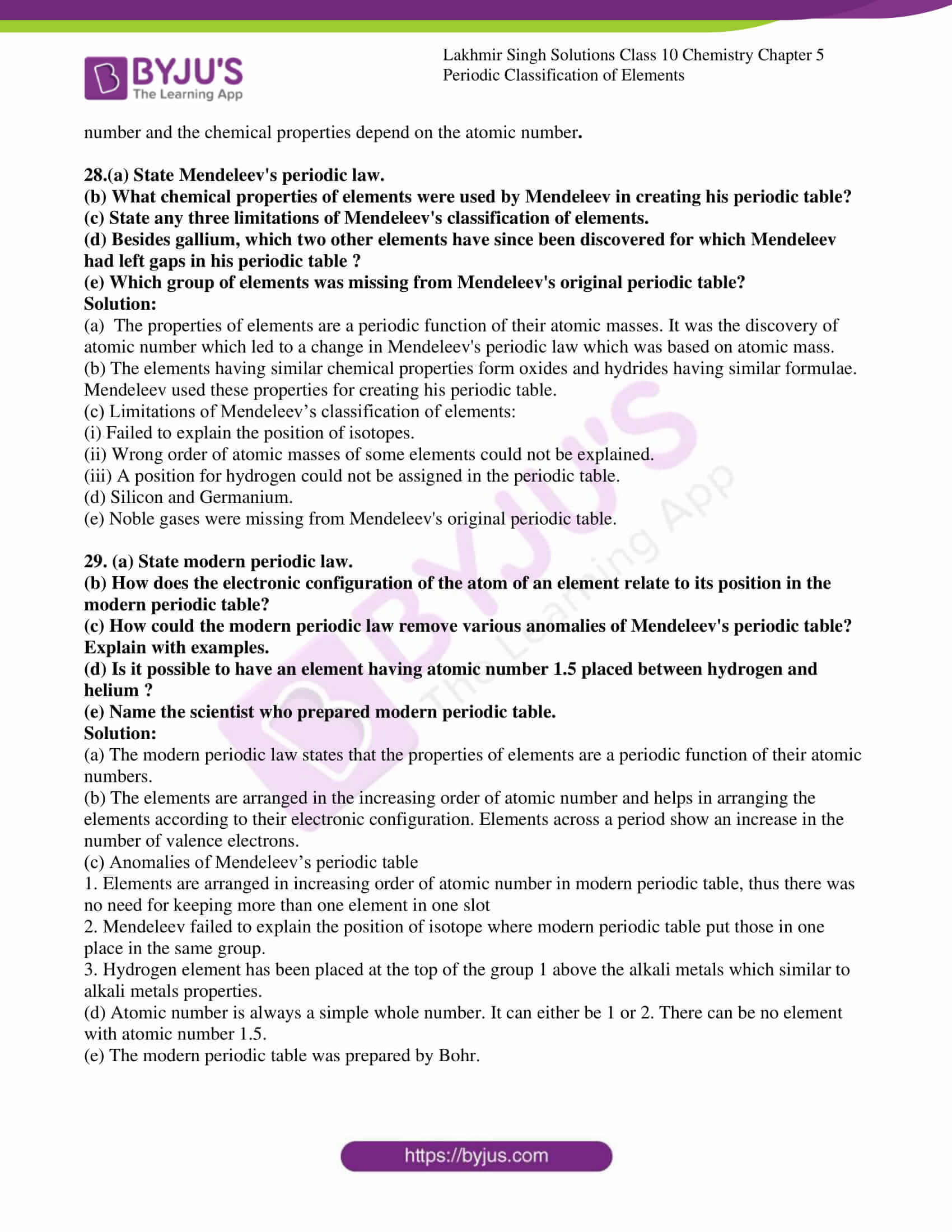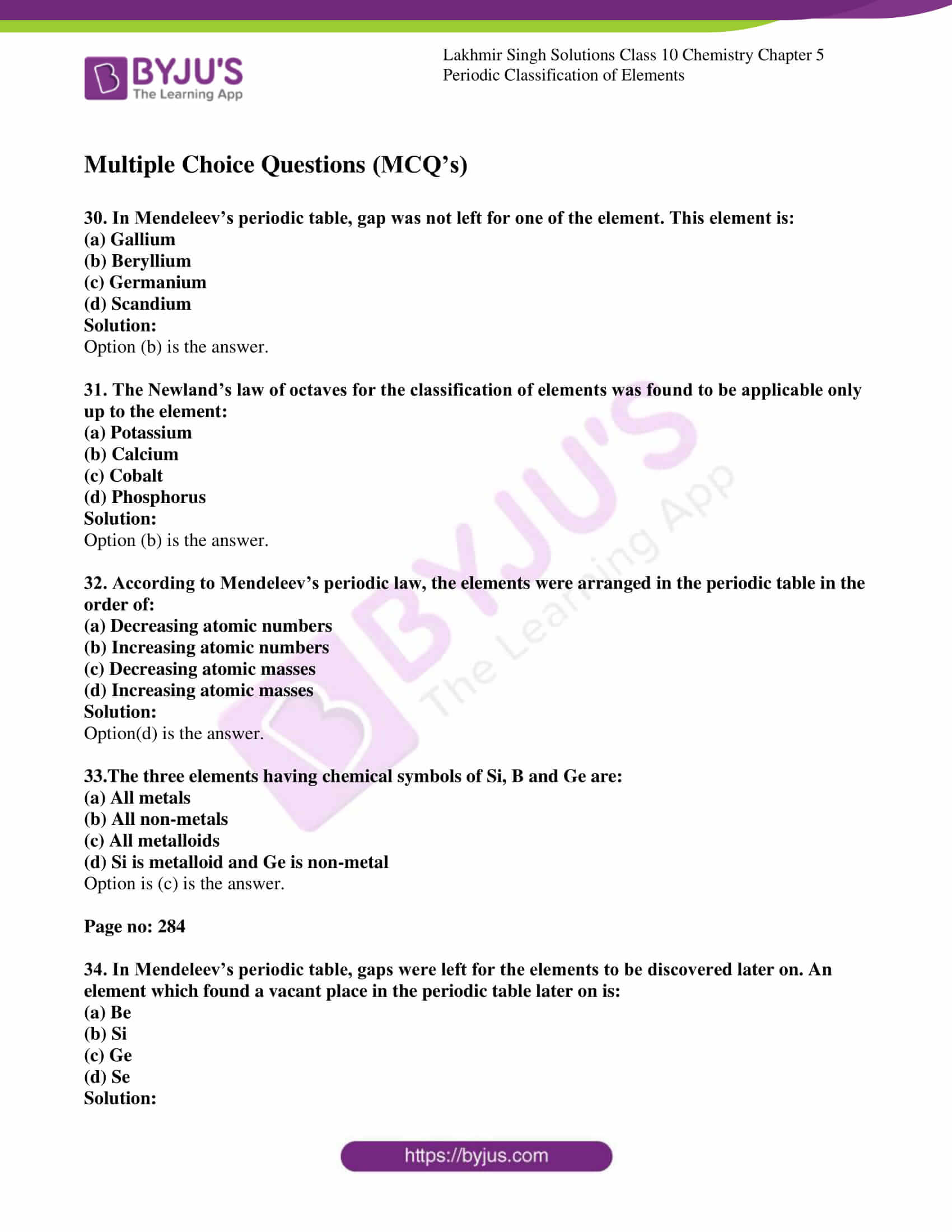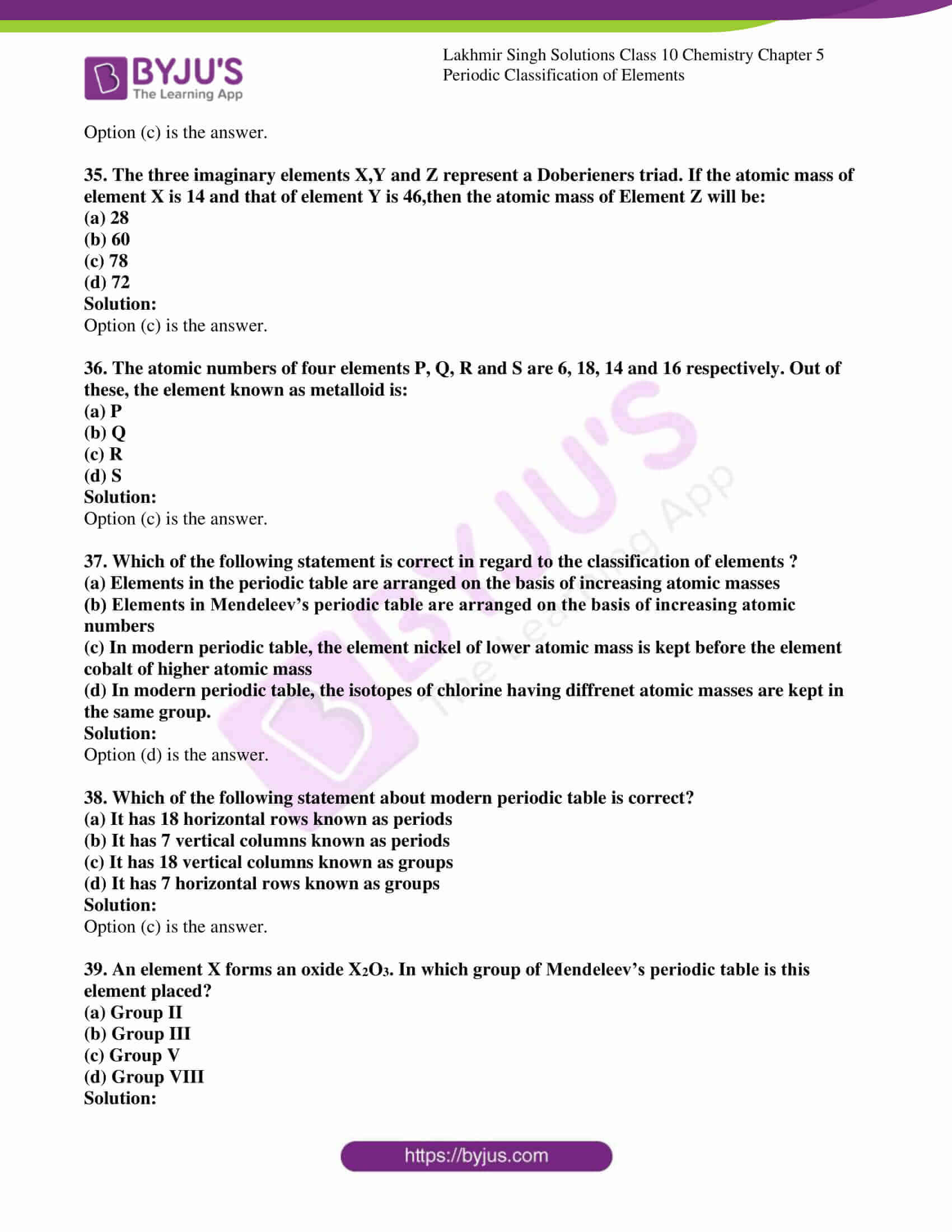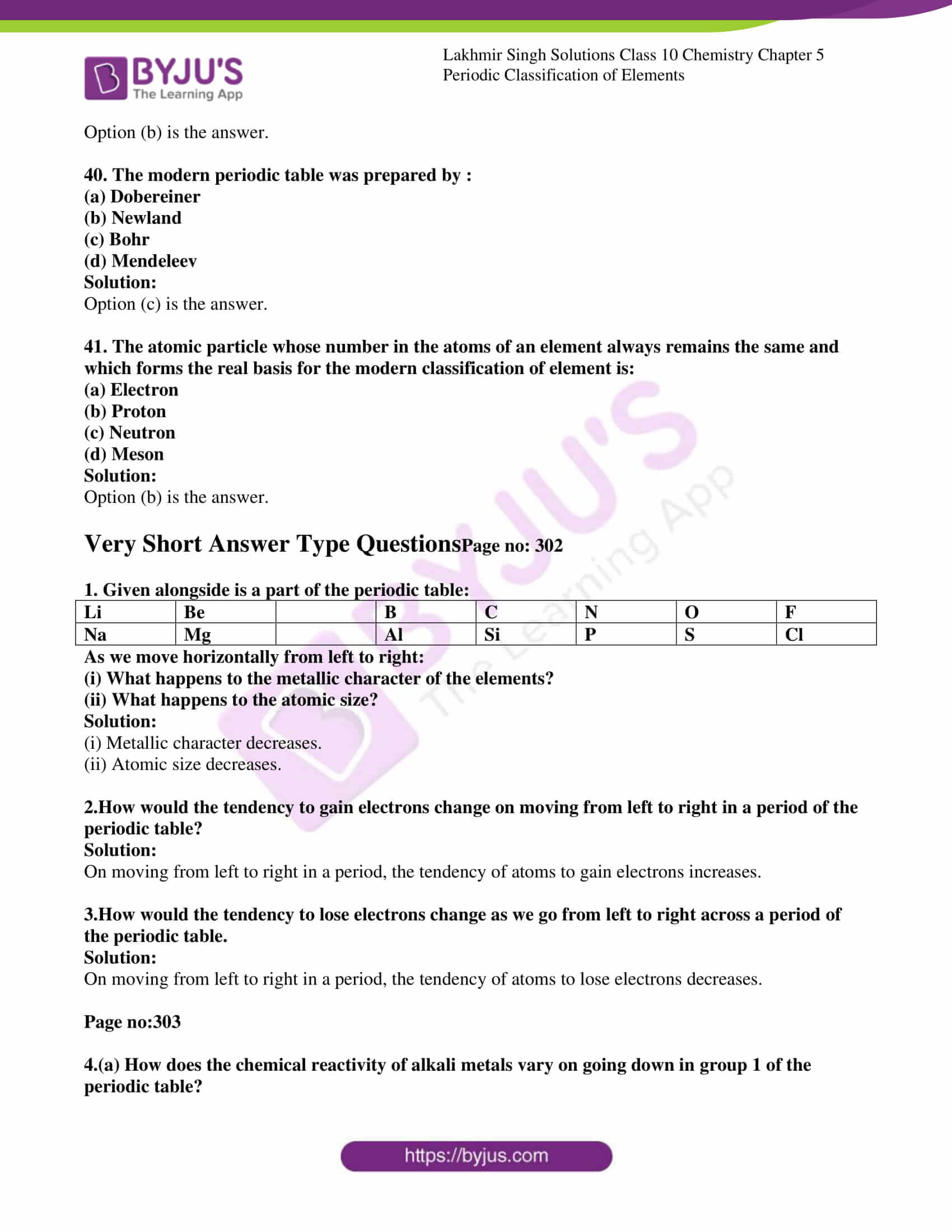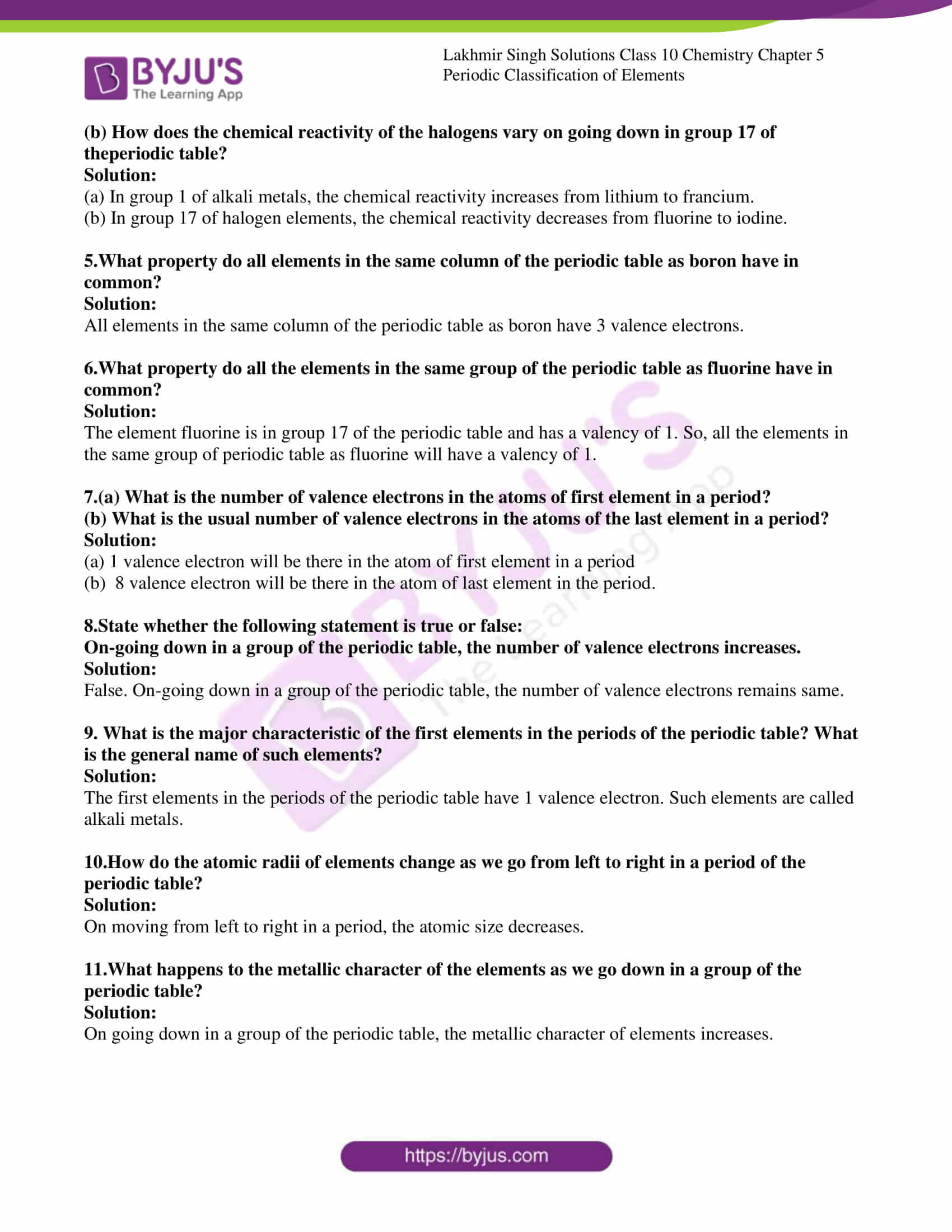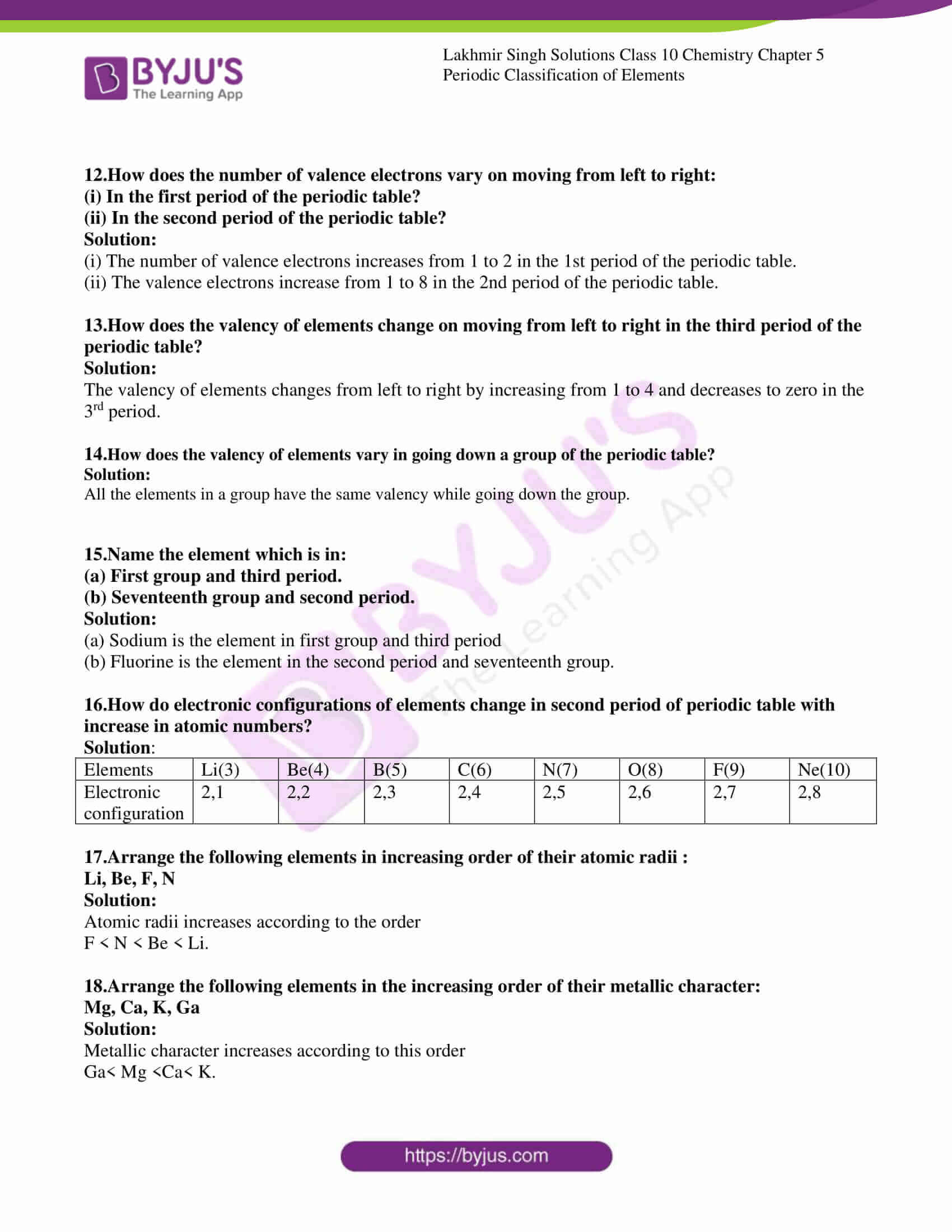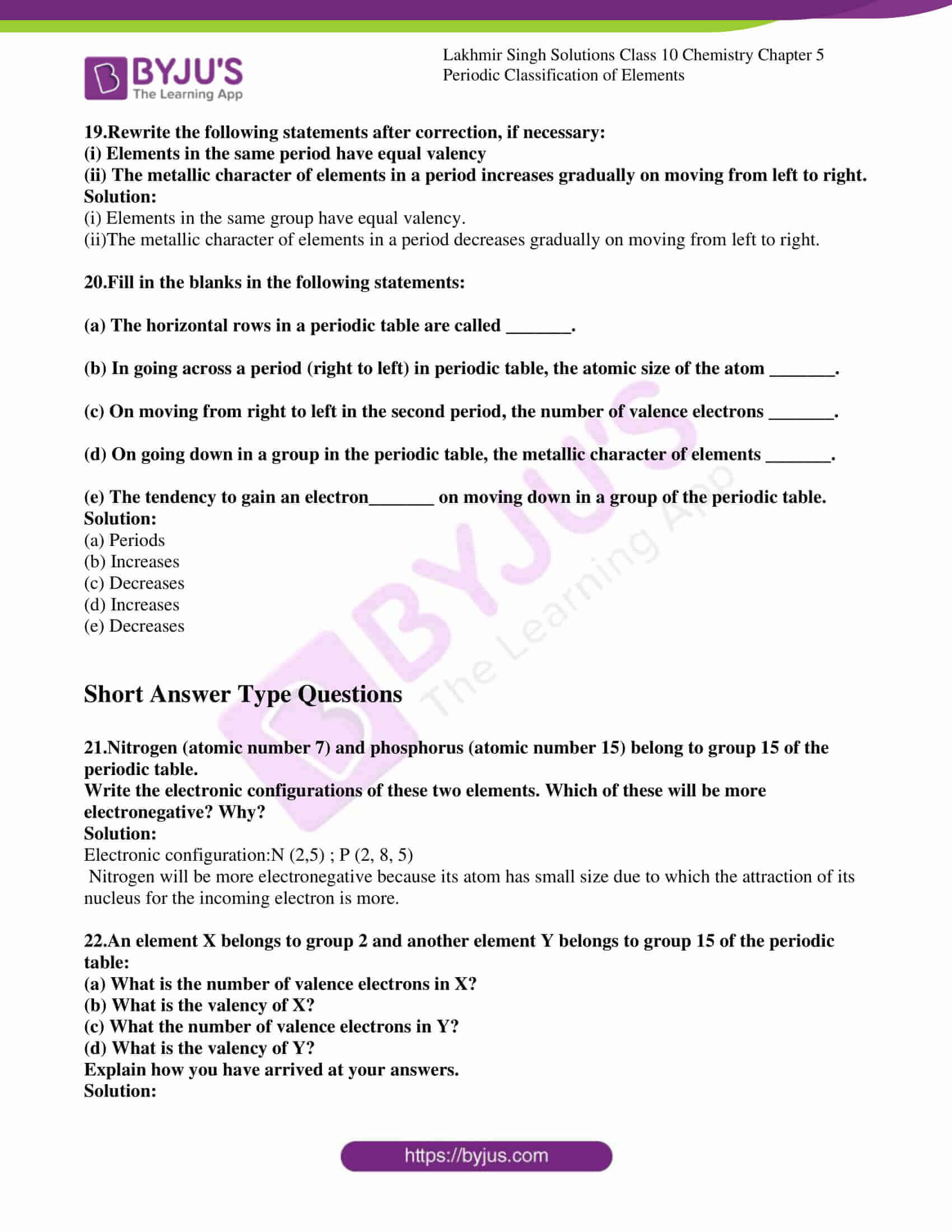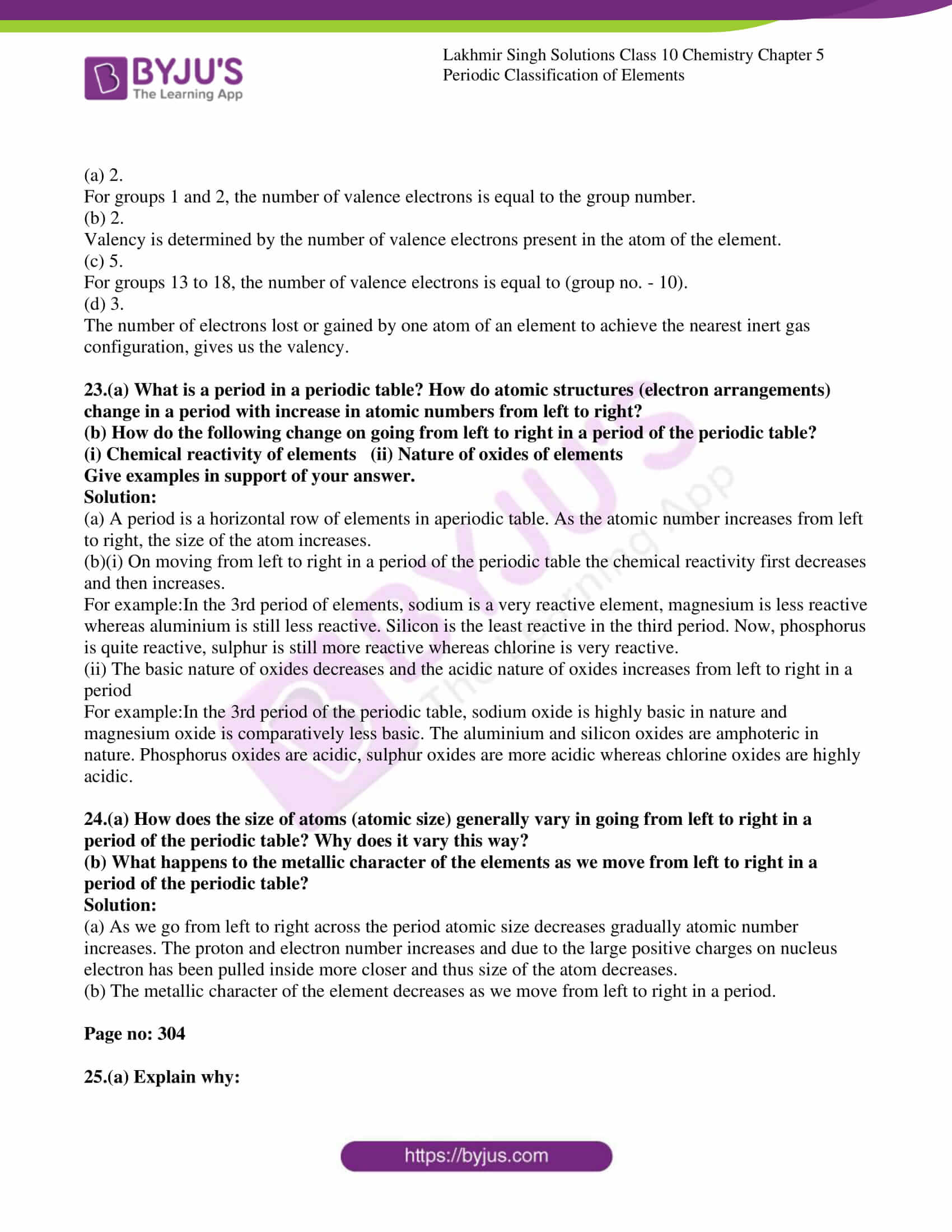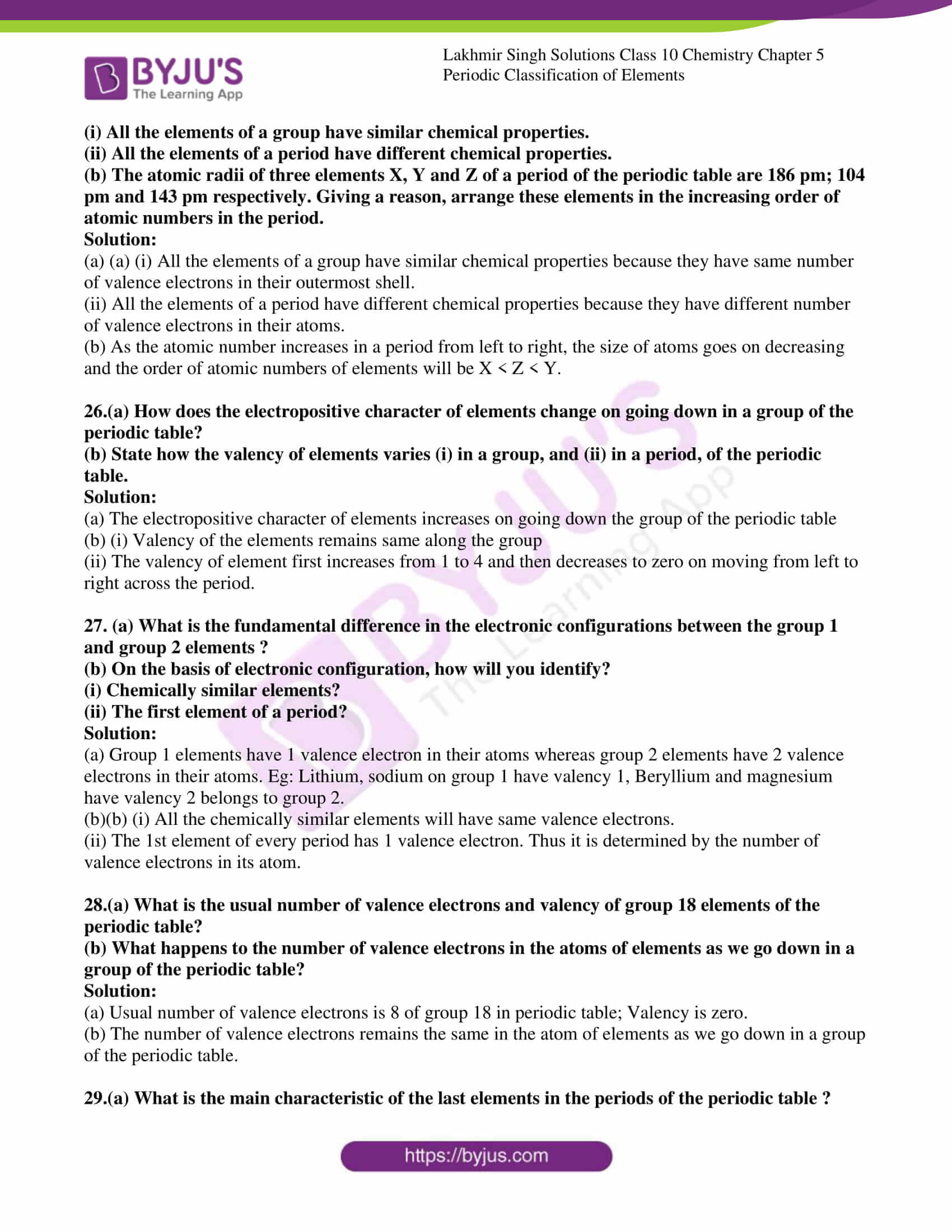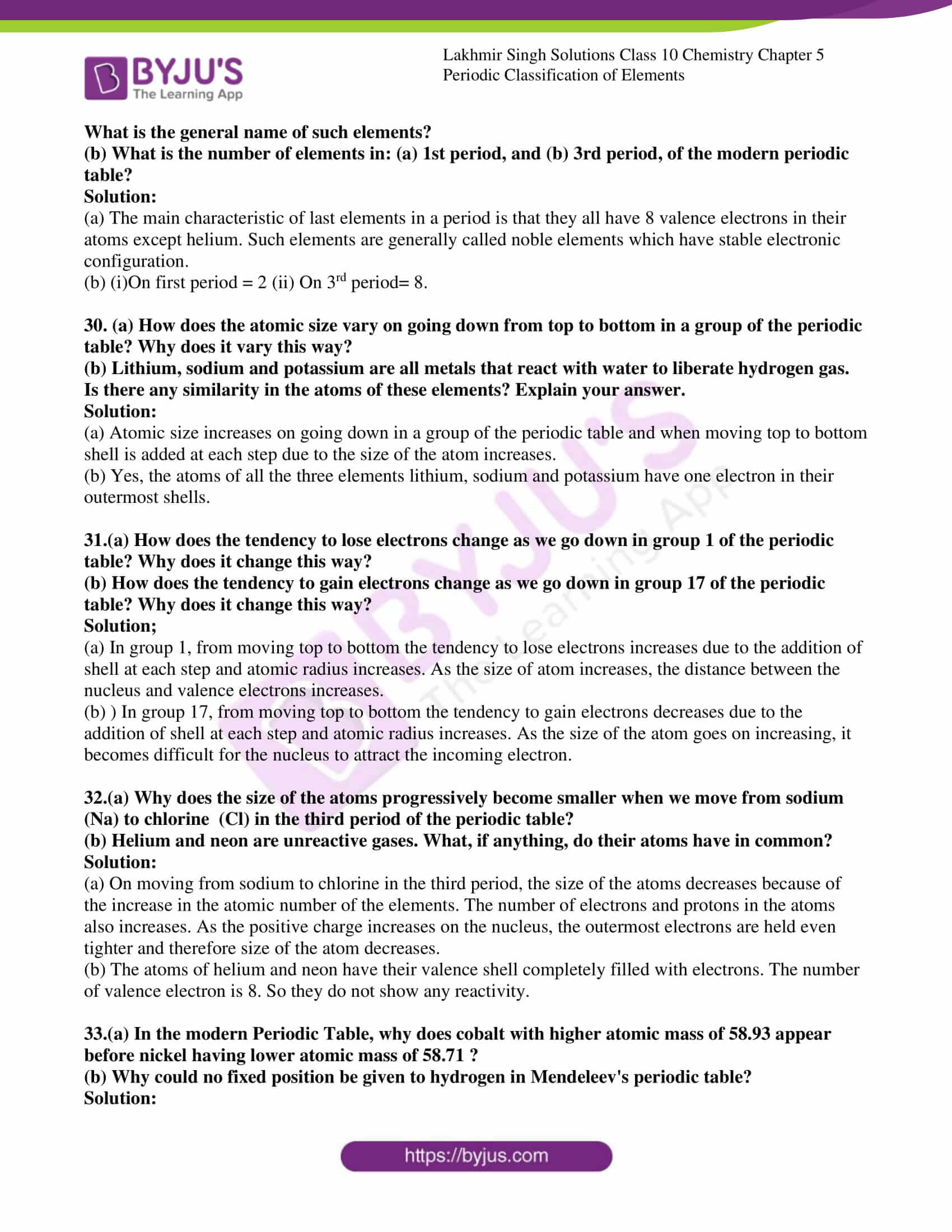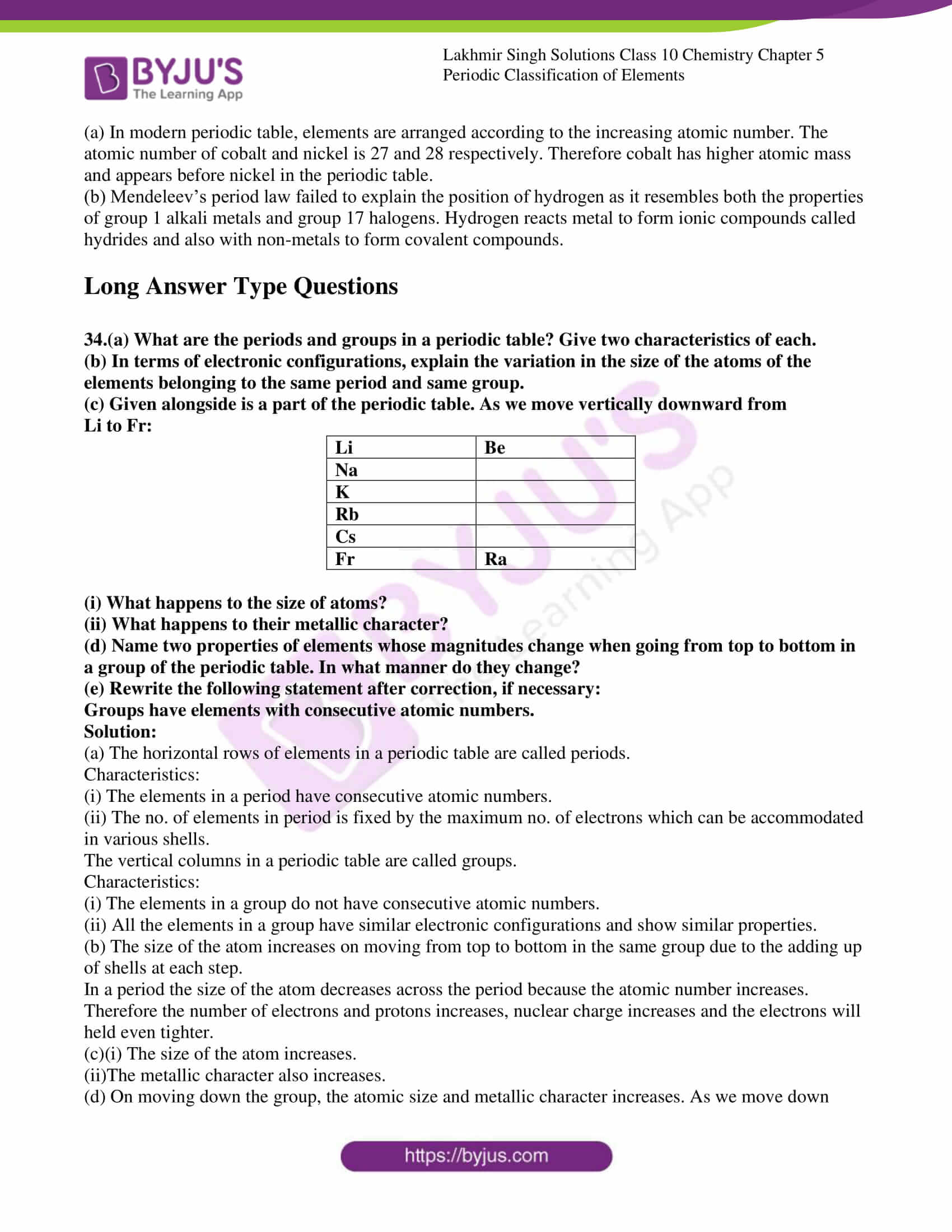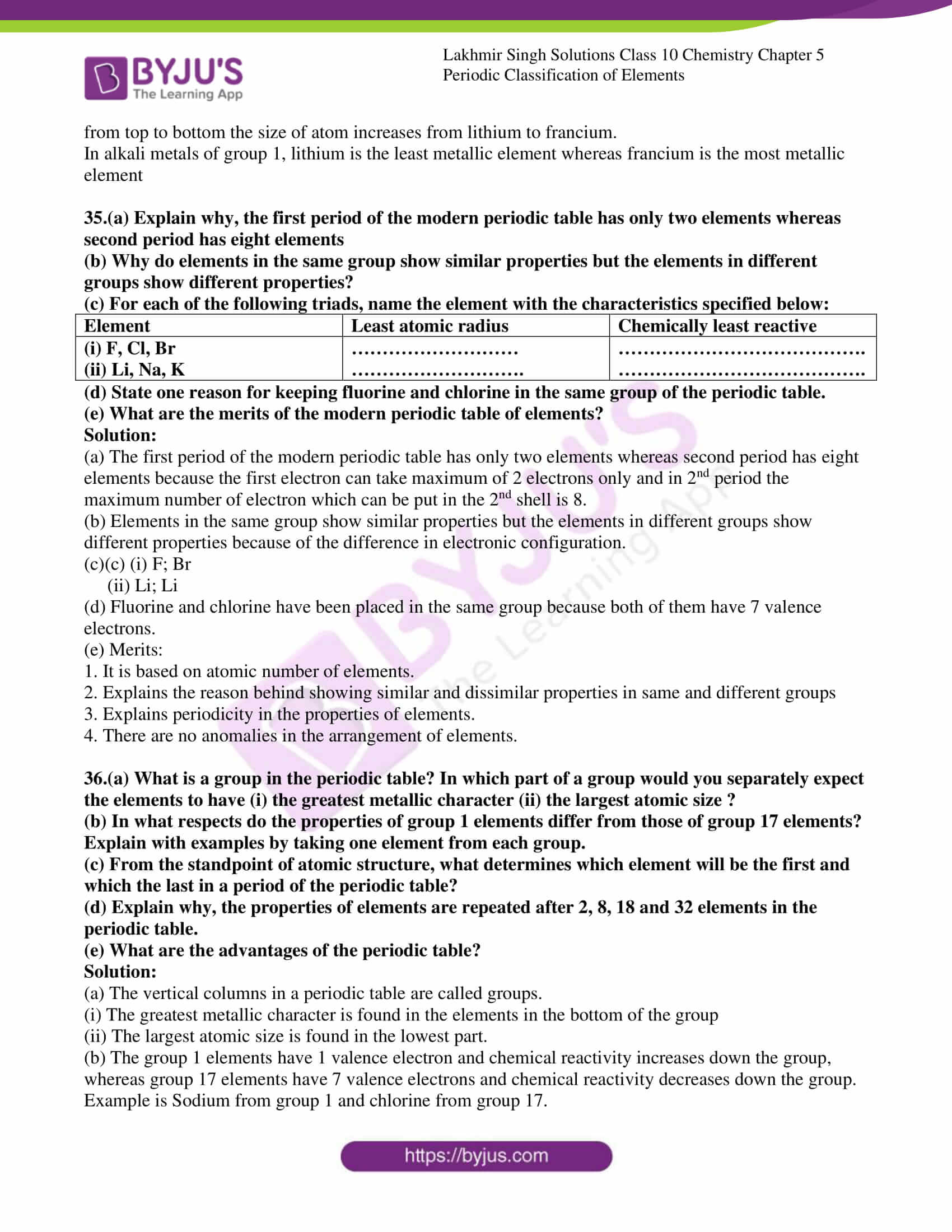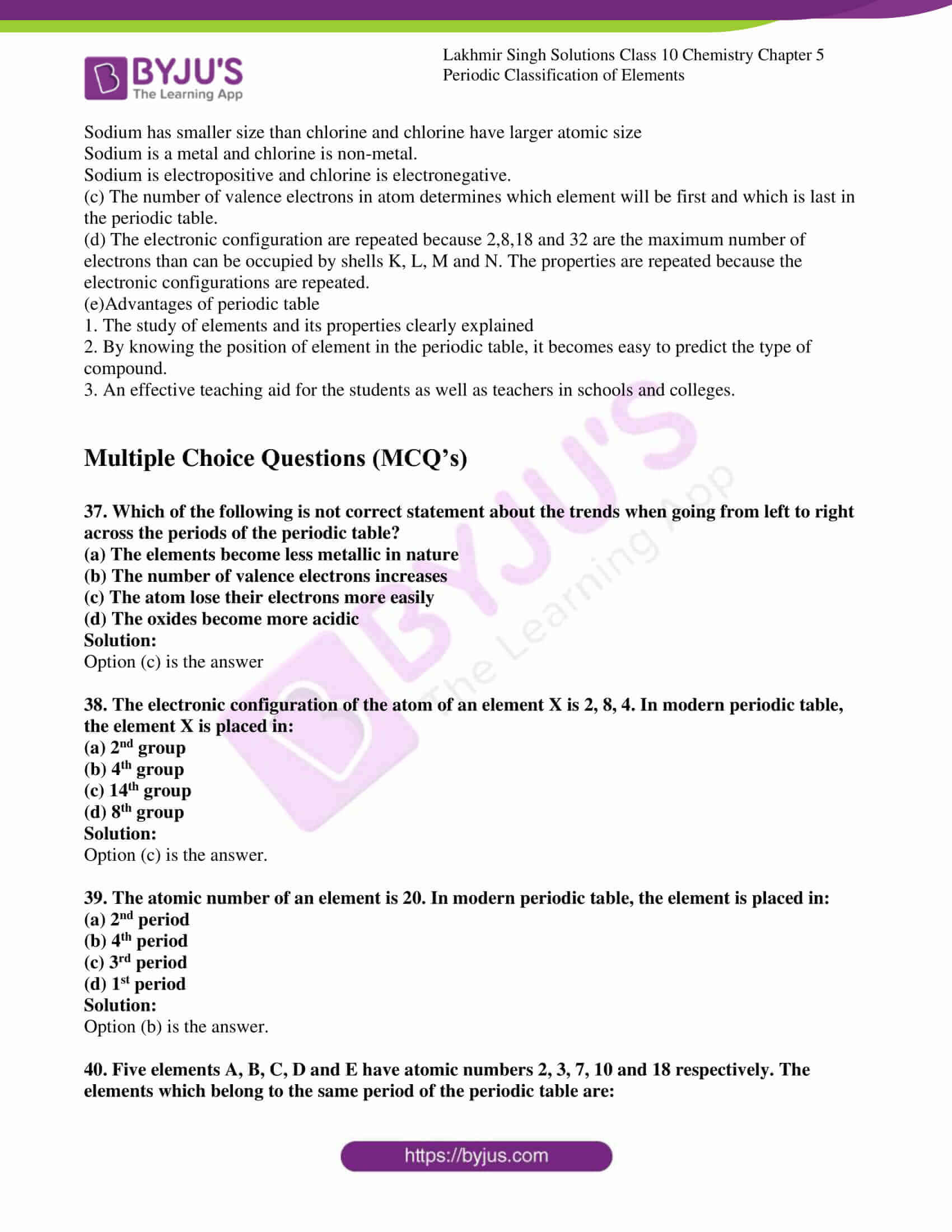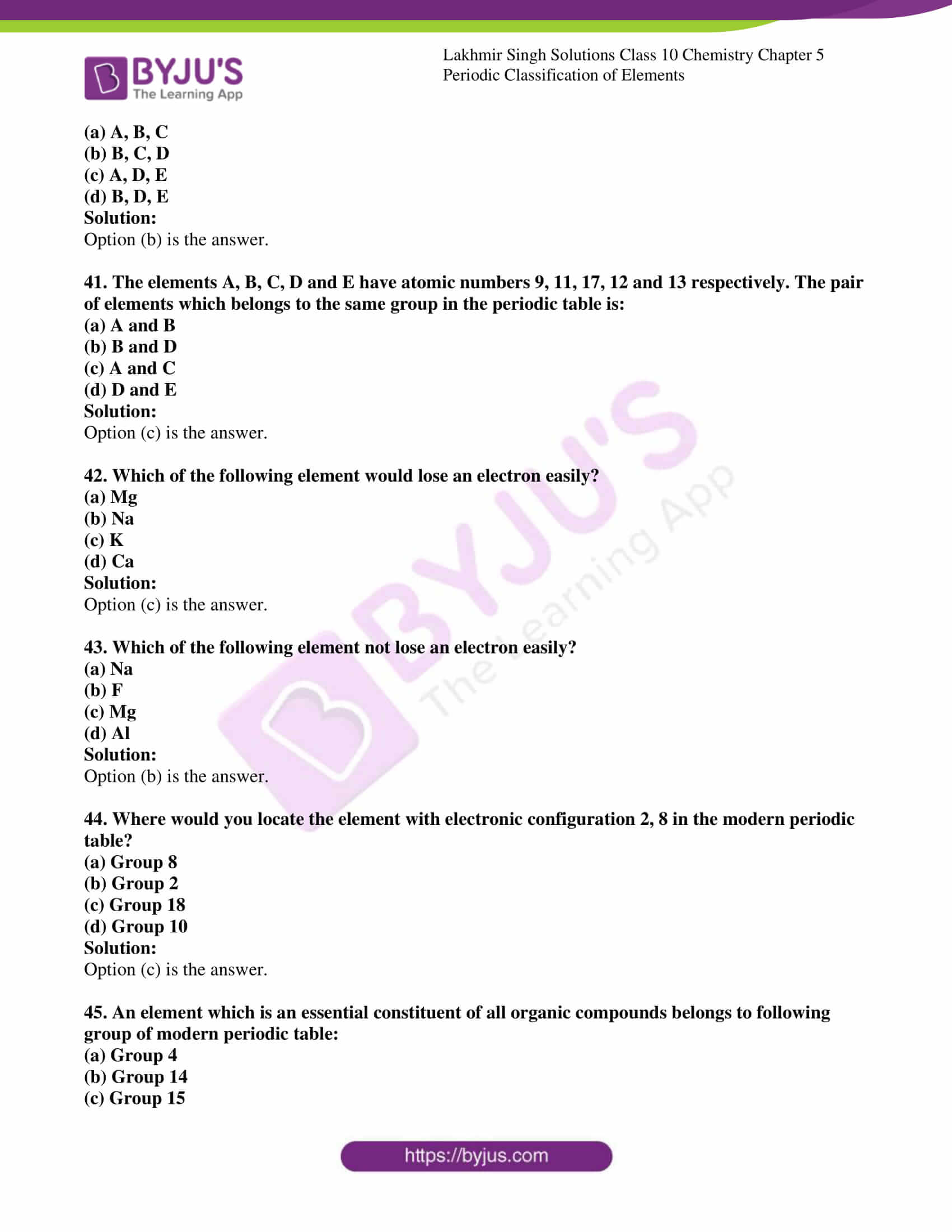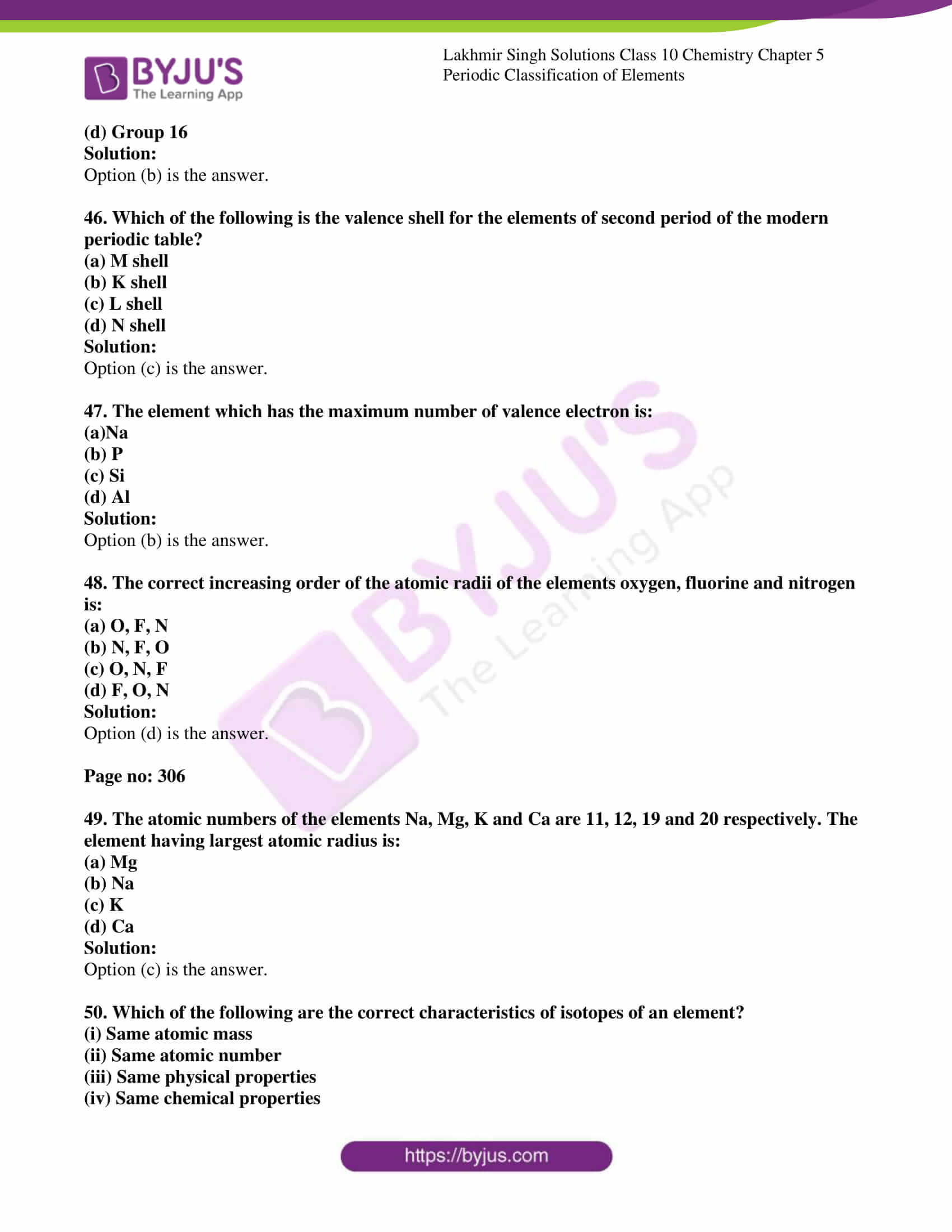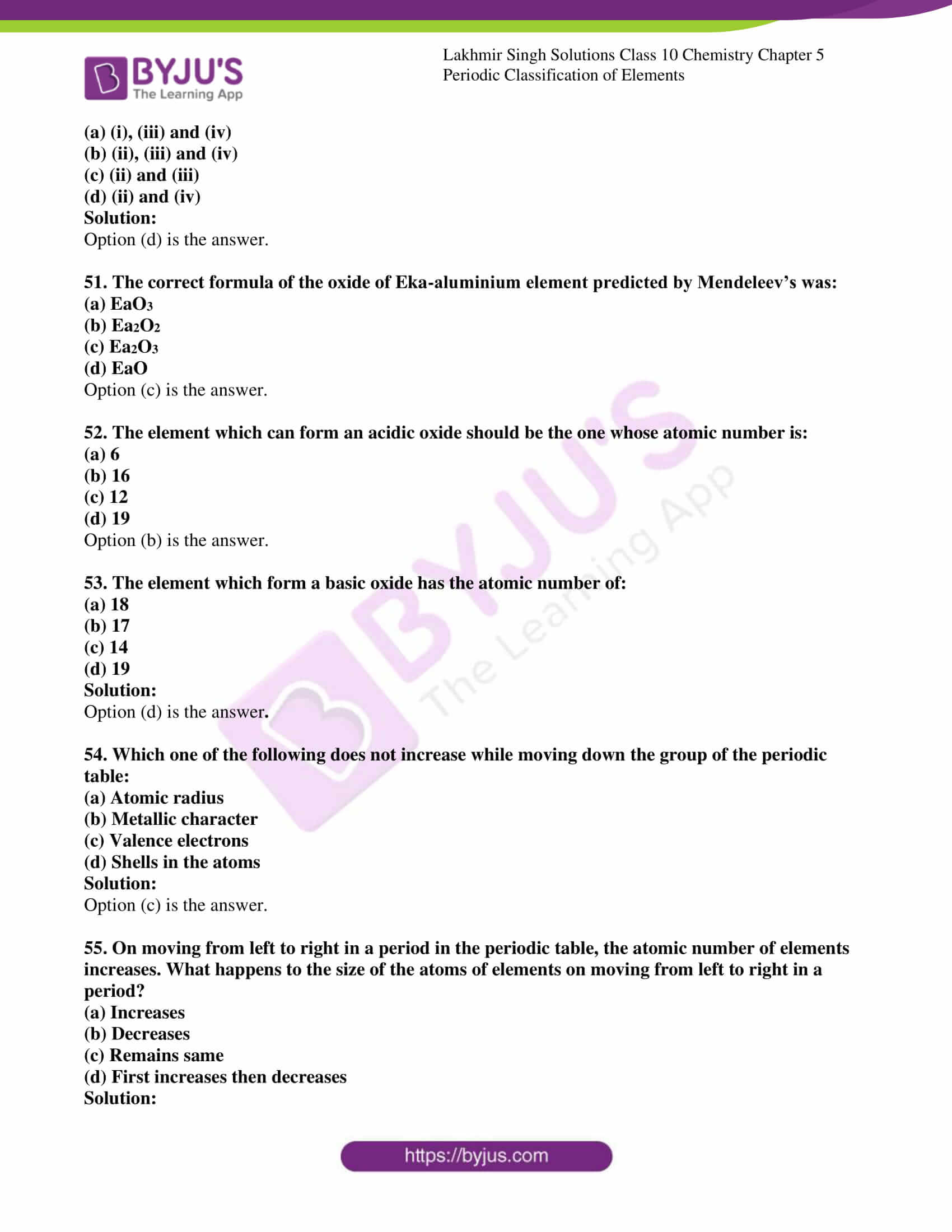### Access Lakhmir Singh Solutions for Class 10 Chemistry Chapter 5

Page no: 281

1. (a) On what basis did Mendeleev arrange the element in his periodic table.

(b) On what basis are they arranged now?

Solution:

(a) On the basis of atomic masses Mendeleev arranged the element in his periodic table.

(b) Now arranged on the basis of atomic numbers.

2.State whether the following statement are true or false:

(a) Newlands divided the elements into horizontal rows of eight element each.

(b) According to Mendeleev’s periodic law, the properties of elements are a periodic function of their atomic numbers.

(c) The elements in a group have consecutive atomic numbers.

Soluti ons:

(a)False. Newlands divided the elements into horizontal rows of seven element each.

(b) False. According to Mendeleev’s periodic law, the properties of elements are a periodic function of their atomic masses

(c) False. The elements in a period have consecutive atomic numbers.

Page no: 282

3.Name the Russian chemist who said that the properties of elements are a periodic function of their atomic masses.

Solution:

Mendeleev said that the properties of elements are a periodic function of their atomic masses.

4.Rewrite the following statements after correction, if necessary:

(a) Groups have elements with consecutive atomic numbers.

(b) Periods are the horizontal rows of elements.

(c) Isotopes are the elements of the same group.

Solution:

(a) Periods have elements with consecutive atomic numbers.

(b) Correct statement

(c) Correct statement

5.Name the scientists who gave the following laws in the early classification of elements:

(a) Law of octaves (b) Law of triads

Solution:

(a) Newlands put forth the law of octaves.

(b) Dobereiner put forth the law of triads.

6.A, B and C are the elements of a Dobereiner’s triad. If the atomic mass of A is 7 and that of C is 39, what should be the atomic mass of B?

Solution:

Mass of A is 7

Mass of C is 39

Mass of B = 7+39/2 = 23

7. X and Y are the two elements having similar properties which obey Newlands’ law of octaves. How many elements are there in-between X and Y?

Solution:

Six elements will be there in between X and Y. Since X and Y are the two elements having similar properties and obey Newlands law of octaves.

8.What was the Mendeleev’s basis for the classification of elements?

Solution:

Mendeleev’s basis for the classification of elements was the atomic mass of elements.

9.In the classification of the then known elements, Mendeleev was guided by two factors. What are those two factors?

Solution:

1. Increasing atomic masses

2. Grouping together of elements having the same properties.

10Name two elements whose properties were predicted on the basis of their positions in Mendeleev’s periodic table.

Solution:

Gallium and Scandium are the two elements whose properties are predicted on the basis of their positions in Mendeleev’s periodic table.

11.The three elements predicted by Mendeleev from the gaps in his periodic table were known as eka-boron, eka – aluminium and eka-silicon. What names were given to these elements when they were discovered later on?

Solution:

Scandium, Gallium, Germanium are the three elements predicted by Mendeleev from the gaps in his periodic table and were known as eka-boron, eka – aluminium and eka-silicon.

12.Name two elements whose properties were predicted on the basis of their positions in Mendeleev’s periodic table.

Solution:

Eka- Aluminium (gallium) and Eka-Silicon (germanium) are the two elements whose properties were predicted on the basis of their positions.

13.State one example of a Dobereiner’s triad, showing in it that the atomic mass of middle element is half-way between those of the other two.

Solution:

Lithiium, sodium and potassium are said to be triads in the Mendeleev’s periodic table. Lithium is the first element in the triad and sodium being middle has atomic mass of 23 and third element is potassium.

Atomic mass of sodium = At. Mass of Li + At. Mass of K / 2

= 7+39/2

=23

14. Which group of elements could be placed in Mendeleev’s periodic table later on, without disturbing the original order? Give reason.

Solution:

Noble gases which are chemically reactive and more stable could be placed in the periodic table in the form of a separate group.

15.Fill in the following blanks with suitable words:

(a) The basis for modern periodic table is ______.

(b) The horizontal rows in a periodic table are called ______.

(c) Group 1 elements are called ______.

(d) Group 17 elements are known as ______.

(e) Group 18 elements are called ______.

(f) According to Newlands’ classification of elements, the properties of sulphur are similar to those of oxygen because sulphur is the ______ element starting from oxygen.

Solution:

(a) Atomic number.

(b) Periods.

(c) Alkali metals.

(d) Halogens.

(e) Noble Gases.

(f) Eighth.

16.(a) What is meant by (i) a group, and (it) a period, in a periodic table?

(b) How many periods and groups are there in the long form of periodic table?

(c) Give two examples each of (i) group 1 elements (ii) group 17 elements (iii) group 18 elements.

Solution:

(a) Groups are the vertical columns in the periodic table

(i) A period is the horizontal rows of elements in the periodic table.

(b) There are seven periods and eighteen groups in the long form of [periodic table.

(c) (i) Group 1: Lithium and Sodium.

(ii) Group 17: Fluorine and Chlorine.

(iii) Group 18: Neon and Argon.

17. (a) In the modem periodic table, which are the metals among the first ten elements?

(b) What is the significance of atomic number in the modern classification of elements? Explain with the help of an example.

Solution:

(a) In modern periodic table, Lithium and beryllium are metals among the first ten elements.

(b) The significance of atomic numbers in the periodic table is the arrangement of elements according to their electronic configuration. The elements placed in same group shows similar chemical properties.

Example: Atomic number of lithium is 3 and its electronic configuration is 2,1. It is placed in first group as the valence electron is 1 and sodium has electronic configuration of 2,8,1 having 1 valence electron and belongs to the same group.

18. (a) How were the positions of isotopes of an element decided in the modern periodic table?

(b) How were the positions of cobalt and nickel resolved in the modern periodic table?

(c) Where should hydrogen be placed in the modern periodic table? Give reason for your answer.

Solution:

(a) Isotopes have same atomic number and different atomic mass, So they are not given separate place in periodic table and is based on the atomic number of elements.

(b) Elements are arranged in the increasing order of their atomic masses as per the Mendeleev’s classification. Cobalt has higher atomic mass was placed before nickel. It has atomic mass of 58.9 and Nickel has 58.7. In modern periodic table, elements are arranged in the increasing order of therir atomic numbers and hence the cobalt and nickel has 27,28 atomic numbers and cobalt with lower atomic number comes before nickel.

(c) Hydrogen is placed at the topmost of periodic table because it resembles both alkali metals and halogens some of the properties so the position in the periodic table is unclear. But it is placed in first group above the alkali metals in the modern periodic table because its electronic configuration is similar to that of alkali metals. As the size is small, it exhibits different properties than of alkali metals.

19.v(a) On which side of the periodic table will you find metals?

(b) On which side of the periodic table will you find non-metals?

(c) What is the name of those elements which divide metals and non-metals in the periodic table?

Solution:

(a) Metals are in left side of the periodic table

(b) Non-metals are in right side of the periodic table

(c) Metalloids is the name of those elements which divide metals and non-metals in periodic table.

Page no:283

20. (a) Name three elements that have a single electron in their outermost shells.

(b) Name two elements that have two electrons in their outermost shells.

(c) Name three elements with completely filled outermost shells.

Solution:

(a) Lithium, Sodium, Potassium are the elements having single electron in their outermost electron.

(b) Magnesium, Calcium has two electrons in the outermost shell

(c) Helium, Neon, Argon are completely filled.

21. What is Dobereiner’s law of triads? Explain with the help of one example of a Dobereiner’s triad.

Solution:

According to Dobereiner’s Law of triads, when elements are arranged in increasing order of their atomic masses, a group of elements with similar chemical properties is obtained. This is called triads. The atomic mass of the middle element is equal to the arithmetic mean of the atomic masses of the other two elements.

For example: Calcium, strontium and barium has atomic masses 40,088 and 137 forms a triad and have similar chemical properties. The atomic mass of strontium is approximately equal to the average of the atomic masses of calcium and barium.

Arithmetic mean of atomic masses of calcium and barium = 40+137/2 = 88.5

22. What is Newlands’ law of octaves? Explain with an example.

Solution:

According to the Newlands’ law of octaves, when elements are arranged in the order of increasing atomic masses, the properties of the eighth element (starting from a given element) are a repetition of the properties of the first element.

For example: Lithium is the first element having valence electron 1 and we find the eighth element from it is sodium having similar properties of lithium.

23.(a) Did Dobereiner’s triads also exist in the columns of Newlands’ law of octaves? Explain your answer.

(b) What were the limitations of Dobereiner’s classification of elements?

(c) What were the limitations of Newlands’ law of octaves?

Solution:

(a) Yes, Dobereiners triads also exist in the columns of Newlands’ Octaves. This can be explained by taking the second column of the Newland’s classification of elements,it has the elements Lithium, Sodium and potassium which form a Dobereiner’s triad.

(b) The main limitation of Dobereiner’s classification of elements was that it failed to arrange all the then known elements in the form of triads of elements having similar chemical properties an also failed to explain the relation between atomic masses of elements and their chemical properties.

(c) (i) It was not applicable throughout the arrangements

(ii) Those elements discovered after Newland’s octaves did not follow the law of octaves.

(iii) The position of cobalt and nickel in the group of elements of different properties could not be explained

(iv)Placing of iron far away from cobalt and nickel, which have similar properties as iron not explained

24. (a) State the periodic law on which Mendeleev’s periodic table was based. Why and how was this periodic law changed ?

(b) Explain why, the noble gases are placed in a separate group.

Solution:

(a) According to Mendeleev’s periodic law: The properties of elements are a periodic function of their atomic masses. It was the discovery of atomic number which led to a change in Mendeleev’s periodic law which was based on atomic mass.

(b) Noble gas are chemically inert or unreactive so they are placed in different group.

25.(a) State the merits of Mendeleev’s classification of elements.

(b) Describe two anomalies of Mendeleev’s periodic classification of elements.

Solution:

(a) Merits;

1. Predicted the existence of certain elements such as gallium, scandium and germanium.

2. Predicted the properties of various elements based on their positions in the periodic table

3. Mendeleev’s periodic table could place group 18 elements called noble gases in the periodic table.

(b)1.This could not explain the position of isotopes having same chemical properties but different atomic masses. So the isotopes of same element should be given different place in periodic table and arranged in increasing order of their atomic masses

2. Mendeleev’s periodic table could not assign a proper place for the element hydrogen

26.(a) How do the properties of eka – aluminium element predicted by Mendeleev compare with the actual properties of gallium element? Explain your answer.

(b) What names were given by Mendeleev to the then undiscovered elements (i) scandium (ii) gallium, and (iii) germanium?

Solution:

(a) Eka-aluminium and gallium are the two different names for a single element. Eka-aluminium has same property like density, melting point etc as gallium element.

(b) (i) Eka boron.

(ii) Eka aluminium.

(iii) Eka-silicon.

27. (a) Why do we classify elements?

(b) What were the two criteria used by Mendeleev to classify the elements in his periodic table?

(c) Why did Mendeleev leave some gaps in his periodic table?

(d) In Mendeleev’s periodic table, why was there no mention of noble gases like helium, neon and argon?

(e) Would you place the two isotopes of chlorine, Cl-35 and Cl-37 in different slots because of their different atomic masses or in the same slot because their chemical properties are the same? Justify your answer.

Solution:

(a) The elements are classified according to the properties possessed by the elements. Similar properties falls in same group and thus large number of elements reduced to a few group and orderly arranged.

(b)(i) Increasing atomic masses

(ii) Grouping together of elements having similar properties.

(c) In order to make sure that the elements having similar properties fell in the same vertical column or group, Mendeleev left some gaps in his periodic table.

(d) The noble gases were not known at that time so there was no mention of noble gases like helium, neon and argon in Mendeleev’s periodic table.

(e) Because their chemical properties are same. The two isotopes of chlorine have the same atomic number and chemical properties. The arrangement of elements is on the basis of increasing atomic number and the chemical properties depend on the atomic number.

28.(a) State Mendeleev’s periodic law.

(b) What chemical properties of elements were used by Mendeleev in creating his periodic table?

(c) State any three limitations of Mendeleev’s classification of elements.

(d) Besides gallium, which two other elements have since been discovered for which Mendeleev had left gaps in his periodic table ?

(e) Which group of elements was missing from Mendeleev’s original periodic table?

Solution:

(a) The properties of elements are a periodic function of their atomic masses. It was the discovery of atomic number which led to a change in Mendeleev’s periodic law which was based on atomic mass.

(b) The elements having similar chemical properties form oxides and hydrides having similar formulae. Mendeleev used these properties for creating his periodic table.

(c) Limitations of Mendeleev’s classification of elements:

(i) Failed to explain the position of isotopes.

(ii) Wrong order of atomic masses of some elements could not be explained.

(iii) A position for hydrogen could not be assigned in the periodic table.

(d) Silicon and Germanium.

(e) Noble gases were missing from Mendeleev’s original periodic table.

29. (a) State modern periodic law.

(b) How does the electronic configuration of the atom of an element relate to its position in the modern periodic table?

(c) How could the modern periodic law remove various anomalies of Mendeleev’s periodic table? Explain with examples.

(d) Is it possible to have an element having atomic number 1.5 placed between hydrogen and helium ?

(e) Name the scientist who prepared modern periodic table.

Solution:

(a) The modern periodic law states that the properties of elements are a periodic function of their atomic numbers.

(b) The elements are arranged in the increasing order of atomic number and helps in arranging the elements according to their electronic configuration. Elements across a period show an increase in the number of valence electrons.

(c) Anomalies of Mendeleev’s periodic table

1. Elements are arranged in increasing order of atomic number in modern periodic table, thus there was no need for keeping more than one element in one slot

2. Mendeleev failed to explain the position of isotope where modern periodic table put those in one place in the same group.

3. Hydrogen element has been placed at the top of the group 1 above the alkali metals which similar to alkali metals properties.

(d) Atomic number is always a simple whole number. It can either be 1 or 2. There can be no element with atomic number 1.5.

(e) The modern periodic table was prepared by Bohr.

Multiple Choice Questions (MCQ’s)

30. In Mendeleev’s periodic table, gap was not left for one of the element. This element is:

(a) Gallium

(b) Beryllium

(c) Germanium

(d) Scandium

Solution:

31. The Newland’s law of octaves for the classification of elements was found to be applicable only up to the element:

(a) Potassium

(b) Calcium

(c) Cobalt

(d) Phosphorus

Solution:

32. According to Mendeleev’s periodic law, the elements were arranged in the periodic table in the order of:

(a) Decreasing atomic numbers

(b) Increasing atomic numbers

(c) Decreasing atomic masses

(d) Increasing atomic masses

Solution:

33.The three elements having chemical symbols of Si, B and Ge are:

(a) All metals

(b) All non-metals

(c) All metalloids

(d) Si is metalloid and Ge is non-metal

Option is (c) is the answer.

Page no: 284

34. In Mendeleev’s periodic table, gaps were left for the elements to be discovered later on. An element which found a vacant place in the periodic table later on is:

(a) Be

(b) Si

(c) Ge

(d) Se

Solution:

35. The three imaginary elements X,Y and Z represent a Doberieners triad. If the atomic mass of element X is 14 and that of element Y is 46,then the atomic mass of Element Z will be:

(a) 28

(b) 60

(c) 78

(d) 72

Solution:

36. The atomic numbers of four elements P, Q, R and S are 6, 18, 14 and 16 respectively. Out of these, the element known as metalloid is:

(a) P

(b) Q

(c) R

(d) S

Solution:

37. Which of the following statement is correct in regard to the classification of elements ?

(a) Elements in the periodic table are arranged on the basis of increasing atomic masses

(b) Elements in Mendeleev’s periodic table are arranged on the basis of increasing atomic numbers

(c) In modern periodic table, the element nickel of lower atomic mass is kept before the element cobalt of higher atomic mass

(d) In modern periodic table, the isotopes of chlorine having diffrenet atomic masses are kept in the same group.

Solution:

38. Which of the following statement about modern periodic table is correct?

(a) It has 18 horizontal rows known as periods

(b) It has 7 vertical columns known as periods

(c) It has 18 vertical columns known as groups

(d) It has 7 horizontal rows known as groups

Solution:

39. An element X forms an oxide X2O3. In which group of Mendeleev’s periodic table is this element placed?

(a) Group II

(b) Group III

(c) Group V

(d) Group VIII

Solution:

40. The modern periodic table was prepared by :

(a) Dobereiner

(b) Newland

(c) Bohr

(d) Mendeleev

Solution:

41. The atomic particle whose number in the atoms of an element always remains the same and which forms the real basis for the modern classification of element is:

(a) Electron

(b) Proton

(c) Neutron

(d) Meson

Solution:

Very Short Answer Type QuestionsPage no: 302

1. Given alongside is a part of the periodic table:

 Li Be B C N O F Na Mg Al Si P S Cl

As we move horizontally from left to right:

(i) What happens to the metallic character of the elements?

(ii) What happens to the atomic size?

Solution:

(i) Metallic character decreases.

(ii) Atomic size decreases.

2.How would the tendency to gain electrons change on moving from left to right in a period of the periodic table?

Solution:

On moving from left to right in a period, the tendency of atoms to gain electrons increases.

3.How would the tendency to lose electrons change as we go from left to right across a period of the periodic table.

Solution:

On moving from left to right in a period, the tendency of atoms to lose electrons decreases.

Page no:303

4.(a) How does the chemical reactivity of alkali metals vary on going down in group 1 of the periodic table?

(b) How does the chemical reactivity of the halogens vary on going down in group 17 of theperiodic table?

Solution:

(a) In group 1 of alkali metals, the chemical reactivity increases from lithium to francium.

(b) In group 17 of halogen elements, the chemical reactivity decreases from fluorine to iodine.

5.What property do all elements in the same column of the periodic table as boron have in common?

Solution:

All elements in the same column of the periodic table as boron have 3 valence electrons.

6.What property do all the elements in the same group of the periodic table as fluorine have in common?

Solution:

The element fluorine is in group 17 of the periodic table and has a valency of 1. So, all the elements in the same group of periodic table as fluorine will have a valency of 1.

7.(a) What is the number of valence electrons in the atoms of first element in a period?

(b) What is the usual number of valence electrons in the atoms of the last element in a period?

Solution:

(a) 1 valence electron will be there in the atom of first element in a period

(b) 8 valence electron will be there in the atom of last element in the period.

8.State whether the following statement is true or false:

On-going down in a group of the periodic table, the number of valence electrons increases.

Solution:

False. On-going down in a group of the periodic table, the number of valence electrons remains same.

9. What is the major characteristic of the first elements in the periods of the periodic table? What is the general name of such elements?

Solution:

The first elements in the periods of the periodic table have 1 valence electron. Such elements are called alkali metals.

10.How do the atomic radii of elements change as we go from left to right in a period of the periodic table?

Solution:

On moving from left to right in a period, the atomic size decreases.

11.What happens to the metallic character of the elements as we go down in a group of the periodic table?

Solution:

On going down in a group of the periodic table, the metallic character of elements increases.

12.How does the number of valence electrons vary on moving from left to right:

(i) In the first period of the periodic table?

(ii) In the second period of the periodic table?

Solution:

(i) The number of valence electrons increases from 1 to 2 in the 1st period of the periodic table.

(ii) The valence electrons increase from 1 to 8 in the 2nd period of the periodic table.

13.How does the valency of elements change on moving from left to right in the third period of the periodic table?

Solution:

The valency of elements changes from left to right by increasing from 1 to 4 and decreases to zero in the 3rd period.

14.How does the valency of elements vary in going down a group of the periodic table?

Solution:

All the elements in a group have the same valency while going down the group.

15.Name the element which is in:

(a) First group and third period.

(b) Seventeenth group and second period.

Solution:

(a) Sodium is the element in first group and third period

(b) Fluorine is the element in the second period and seventeenth group.

16.How do electronic configurations of elements change in second period of periodic table with increase in atomic numbers?

Solution:

 Elements Li(3) Be(4) B(5) C(6) N(7) O(8) F(9) Ne(10) Electronic configuration 2,1 2,2 2,3 2,4 2,5 2,6 2,7 2,8

17.Arrange the following elements in increasing order of their atomic radii :

Li, Be, F, N

Solution:

Atomic radii increases according to the order

F < N < Be < Li.

18.Arrange the following elements in the increasing order of their metallic character:

Mg, Ca, K, Ga

Solution:

Metallic character increases according to this order

Ga< Mg <Ca< K.

19.Rewrite the following statements after correction, if necessary:

(i) Elements in the same period have equal valency

(ii) The metallic character of elements in a period increases gradually on moving from left to right.

Solution:

(i) Elements in the same group have equal valency.

(ii)The metallic character of elements in a period decreases gradually on moving from left to right.

20.Fill in the blanks in the following statements:

(a) The horizontal rows in a periodic table are called _______.

(b) In going across a period (right to left) in periodic table, the atomic size of the atom _______.

(c) On moving from right to left in the second period, the number of valence electrons _______.

(d) On going down in a group in the periodic table, the metallic character of elements _______.

(e) The tendency to gain an electron_______ on moving down in a group of the periodic table.

Solution:

(a) Periods

(b) Increases

(c) Decreases

(d) Increases

(e) Decreases

21.Nitrogen (atomic number 7) and phosphorus (atomic number 15) belong to group 15 of the periodic table.

Write the electronic configurations of these two elements. Which of these will be more electronegative? Why?

Solution:

Electronic configuration:N (2,5) ; P (2, 8, 5)

Nitrogen will be more electronegative because its atom has small size due to which the attraction of its nucleus for the incoming electron is more.

22.An element X belongs to group 2 and another element Y belongs to group 15 of the periodic table:

(a) What is the number of valence electrons in X?

(b) What is the valency of X?

(c) What the number of valence electrons in Y?

(d) What is the valency of Y?

Solution:

(a) 2.

For groups 1 and 2, the number of valence electrons is equal to the group number.

(b) 2.

Valency is determined by the number of valence electrons present in the atom of the element.

(c) 5.

For groups 13 to 18, the number of valence electrons is equal to (group no. – 10).

(d) 3.

The number of electrons lost or gained by one atom of an element to achieve the nearest inert gas configuration, gives us the valency.

23.(a) What is a period in a periodic table? How do atomic structures (electron arrangements) change in a period with increase in atomic numbers from left to right?

(b) How do the following change on going from left to right in a period of the periodic table?

(i) Chemical reactivity of elements (ii) Nature of oxides of elements

Solution:

(a) A period is a horizontal row of elements in aperiodic table. As the atomic number increases from left to right, the size of the atom increases.

(b)(i) On moving from left to right in a period of the periodic table the chemical reactivity first decreases and then increases.

For example:In the 3rd period of elements, sodium is a very reactive element, magnesium is less reactive whereas aluminium is still less reactive. Silicon is the least reactive in the third period. Now, phosphorus is quite reactive, sulphur is still more reactive whereas chlorine is very reactive.

(ii) The basic nature of oxides decreases and the acidic nature of oxides increases from left to right in a period

For example:In the 3rd period of the periodic table, sodium oxide is highly basic in nature and magnesium oxide is comparatively less basic. The aluminium and silicon oxides are amphoteric in nature. Phosphorus oxides are acidic, sulphur oxides are more acidic whereas chlorine oxides are highly acidic.

24.(a) How does the size of atoms (atomic size) generally vary in going from left to right in a period of the periodic table? Why does it vary this way?

(b) What happens to the metallic character of the elements as we move from left to right in a period of the periodic table?

Solution:

(a) As we go from left to right across the period atomic size decreases gradually atomic number increases. The proton and electron number increases and due to the large positive charges on nucleus electron has been pulled inside more closer and thus size of the atom decreases.

(b) The metallic character of the element decreases as we move from left to right in a period.

Page no: 304

25.(a) Explain why:

(i) All the elements of a group have similar chemical properties.

(ii) All the elements of a period have different chemical properties.

(b) The atomic radii of three elements X, Y and Z of a period of the periodic table are 186 pm; 104 pm and 143 pm respectively. Giving a reason, arrange these elements in the increasing order of atomic numbers in the period.

Solution:

(a) (a) (i) All the elements of a group have similar chemical properties because they have same number of valence electrons in their outermost shell.

(ii) All the elements of a period have different chemical properties because they have different number of valence electrons in their atoms.

(b) As the atomic number increases in a period from left to right, the size of atoms goes on decreasing and the order of atomic numbers of elements will be X < Z < Y.

26.(a) How does the electropositive character of elements change on going down in a group of the periodic table?

(b) State how the valency of elements varies (i) in a group, and (ii) in a period, of the periodic table.

Solution:

(a) The electropositive character of elements increases on going down the group of the periodic table

(b) (i) Valency of the elements remains same along the group

(ii) The valency of element first increases from 1 to 4 and then decreases to zero on moving from left to right across the period.

27. (a) What is the fundamental difference in the electronic configurations between the group 1 and group 2 elements ?

(b) On the basis of electronic configuration, how will you identify?

(i) Chemically similar elements?

(ii) The first element of a period?

Solution:

(a) Group 1 elements have 1 valence electron in their atoms whereas group 2 elements have 2 valence electrons in their atoms. Eg: Lithium, sodium on group 1 have valency 1, Beryllium and magnesium have valency 2 belongs to group 2.

(b)(b) (i) All the chemically similar elements will have same valence electrons.

(ii) The 1st element of every period has 1 valence electron. Thus it is determined by the number of valence electrons in its atom.

28.(a) What is the usual number of valence electrons and valency of group 18 elements of the periodic table?

(b) What happens to the number of valence electrons in the atoms of elements as we go down in a group of the periodic table?

Solution:

(a) Usual number of valence electrons is 8 of group 18 in periodic table; Valency is zero.

(b) The number of valence electrons remains the same in the atom of elements as we go down in a group of the periodic table.

29.(a) What is the main characteristic of the last elements in the periods of the periodic table ? What is the general name of such elements?

(b) What is the number of elements in: (a) 1st period, and (b) 3rd period, of the modern periodic table?

Solution:

(a) The main characteristic of last elements in a period is that they all have 8 valence electrons in their atoms except helium. Such elements are generally called noble elements which have stable electronic configuration.

(b) (i)On first period = 2 (ii) On 3rd period= 8.

30. (a) How does the atomic size vary on going down from top to bottom in a group of the periodic table? Why does it vary this way?

(b) Lithium, sodium and potassium are all metals that react with water to liberate hydrogen gas. Is there any similarity in the atoms of these elements? Explain your answer.

Solution:

(a) Atomic size increases on going down in a group of the periodic table and when moving top to bottom shell is added at each step due to the size of the atom increases.

(b) Yes, the atoms of all the three elements lithium, sodium and potassium have one electron in their outermost shells.

31.(a) How does the tendency to lose electrons change as we go down in group 1 of the periodic table? Why does it change this way?

(b) How does the tendency to gain electrons change as we go down in group 17 of the periodic table? Why does it change this way?

Solution;

(a) In group 1, from moving top to bottom the tendency to lose electrons increases due to the addition of shell at each step and atomic radius increases. As the size of atom increases, the distance between the nucleus and valence electrons increases.

(b) ) In group 17, from moving top to bottom the tendency to gain electrons decreases due to the addition of shell at each step and atomic radius increases. As the size of the atom goes on increasing, it becomes difficult for the nucleus to attract the incoming electron.

32.(a) Why does the size of the atoms progressively become smaller when we move from sodium (Na) to chlorine (Cl) in the third period of the periodic table?

(b) Helium and neon are unreactive gases. What, if anything, do their atoms have in common?

Solution:

(a) On moving from sodium to chlorine in the third period, the size of the atoms decreases because of the increase in the atomic number of the elements. The number of electrons and protons in the atoms also increases. As the positive charge increases on the nucleus, the outermost electrons are held even tighter and therefore size of the atom decreases.

(b) The atoms of helium and neon have their valence shell completely filled with electrons. The number of valence electron is 8. So they do not show any reactivity.

33.(a) In the modern Periodic Table, why does cobalt with higher atomic mass of 58.93 appear before nickel having lower atomic mass of 58.71 ?

(b) Why could no fixed position be given to hydrogen in Mendeleev’s periodic table?

Solution:

(a) In modern periodic table, elements are arranged according to the increasing atomic number. The atomic number of cobalt and nickel is 27 and 28 respectively. Therefore cobalt has higher atomic mass and appears before nickel in the periodic table.

(b) Mendeleev’s period law failed to explain the position of hydrogen as it resembles both the properties of group 1 alkali metals and group 17 halogens. Hydrogen reacts metal to form ionic compounds called hydrides and also with non-metals to form covalent compounds.

34.(a) What are the periods and groups in a periodic table? Give two characteristics of each.

(b) In terms of electronic configurations, explain the variation in the size of the atoms of the elements belonging to the same period and same group.

(c) Given alongside is a part of the periodic table. As we move vertically downward from

Li to Fr:

 Li Be Na K Rb Cs Fr Ra

(i) What happens to the size of atoms?

(ii) What happens to their metallic character?

(d) Name two properties of elements whose magnitudes change when going from top to bottom in a group of the periodic table. In what manner do they change?

(e) Rewrite the following statement after correction, if necessary:

Groups have elements with consecutive atomic numbers.

Solution:

(a) The horizontal rows of elements in a periodic table are called periods.

Characteristics:

(i) The elements in a period have consecutive atomic numbers.

(ii) The no. of elements in period is fixed by the maximum no. of electrons which can be accommodated in various shells.

The vertical columns in a periodic table are called groups.

Characteristics:

(i) The elements in a group do not have consecutive atomic numbers.

(ii) All the elements in a group have similar electronic configurations and show similar properties.

(b) The size of the atom increases on moving from top to bottom in the same group due to the adding up of shells at each step.

In a period the size of the atom decreases across the period because the atomic number increases. Therefore the number of electrons and protons increases, nuclear charge increases and the electrons will held even tighter.

(c)(i) The size of the atom increases.

(ii)The metallic character also increases.

(d) On moving down the group, the atomic size and metallic character increases. As we move down from top to bottom the size of atom increases from lithium to francium.

In alkali metals of group 1, lithium is the least metallic element whereas francium is the most metallic element

35.(a) Explain why, the first period of the modern periodic table has only two elements whereas second period has eight elements

(b) Why do elements in the same group show similar properties but the elements in different groups show different properties?

(c) For each of the following triads, name the element with the characteristics specified below:

 Element Least atomic radius Chemically least reactive (i) F, Cl, Br (ii) Li, Na, K ……………………… ………………………. …………………………………. ………………………………….

(d) State one reason for keeping fluorine and chlorine in the same group of the periodic table.

(e) What are the merits of the modern periodic table of elements?

Solution:

(a) The first period of the modern periodic table has only two elements whereas second period has eight elements because the first electron can take maximum of 2 electrons only and in 2nd period the maximum number of electron which can be put in the 2nd shell is 8.

(b) Elements in the same group show similar properties but the elements in different groups show different properties because of the difference in electronic configuration.

(c)(c) (i) F; Br

(ii) Li; Li

(d) Fluorine and chlorine have been placed in the same group because both of them have 7 valence electrons.

(e) Merits:

1. It is based on atomic number of elements.

2. Explains the reason behind showing similar and dissimilar properties in same and different groups

3. Explains periodicity in the properties of elements.

4. There are no anomalies in the arrangement of elements.

36.(a) What is a group in the periodic table? In which part of a group would you separately expect the elements to have (i) the greatest metallic character (ii) the largest atomic size ?

(b) In what respects do the properties of group 1 elements differ from those of group 17 elements? Explain with examples by taking one element from each group.

(c) From the standpoint of atomic structure, what determines which element will be the first and which the last in a period of the periodic table?

(d) Explain why, the properties of elements are repeated after 2, 8, 18 and 32 elements in the periodic table.

(e) What are the advantages of the periodic table?

Solution:

(a) The vertical columns in a periodic table are called groups.

(i) The greatest metallic character is found in the elements in the bottom of the group

(ii) The largest atomic size is found in the lowest part.

(b) The group 1 elements have 1 valence electron and chemical reactivity increases down the group, whereas group 17 elements have 7 valence electrons and chemical reactivity decreases down the group.

Example is Sodium from group 1 and chlorine from group 17.

Sodium has smaller size than chlorine and chlorine have larger atomic size

Sodium is a metal and chlorine is non-metal.

Sodium is electropositive and chlorine is electronegative.

(c) The number of valence electrons in atom determines which element will be first and which is last in the periodic table.

(d) The electronic configuration are repeated because 2,8,18 and 32 are the maximum number of electrons than can be occupied by shells K, L, M and N. The properties are repeated because the electronic configurations are repeated.

1. The study of elements and its properties clearly explained

2. By knowing the position of element in the periodic table, it becomes easy to predict the type of compound.

3. An effective teaching aid for the students as well as teachers in schools and colleges.

Multiple Choice Questions (MCQ’s)

37. Which of the following is not correct statement about the trends when going from left to right across the periods of the periodic table?

(a) The elements become less metallic in nature

(b) The number of valence electrons increases

(c) The atom lose their electrons more easily

(d) The oxides become more acidic

Solution:

38. The electronic configuration of the atom of an element X is 2, 8, 4. In modern periodic table, the element X is placed in:

(a) 2nd group

(b) 4th group

(c) 14th group

(d) 8th group

Solution:

39. The atomic number of an element is 20. In modern periodic table, the element is placed in:

(a) 2nd period

(b) 4th period

(c) 3rd period

(d) 1st period

Solution:

40. Five elements A, B, C, D and E have atomic numbers 2, 3, 7, 10 and 18 respectively. The elements which belong to the same period of the periodic table are:

(a) A, B, C

(b) B, C, D

(c) A, D, E

(d) B, D, E

Solution:

41. The elements A, B, C, D and E have atomic numbers 9, 11, 17, 12 and 13 respectively. The pair of elements which belongs to the same group in the periodic table is:

(a) A and B

(b) B and D

(c) A and C

(d) D and E

Solution:

42. Which of the following element would lose an electron easily?

(a) Mg

(b) Na

(c) K

(d) Ca

Solution:

43. Which of the following element not lose an electron easily?

(a) Na

(b) F

(c) Mg

(d) Al

Solution:

44. Where would you locate the element with electronic configuration 2, 8 in the modern periodic table?

(a) Group 8

(b) Group 2

(c) Group 18

(d) Group 10

Solution:

45. An element which is an essential constituent of all organic compounds belongs to following group of modern periodic table:

(a) Group 4

(b) Group 14

(c) Group 15

(d) Group 16

Solution:

46. Which of the following is the valence shell for the elements of second period of the modern periodic table?

(a) M shell

(b) K shell

(c) L shell

(d) N shell

Solution:

47. The element which has the maximum number of valence electron is:

(a)Na

(b) P

(c) Si

(d) Al

Solution:

48. The correct increasing order of the atomic radii of the elements oxygen, fluorine and nitrogen is:

(a) O, F, N

(b) N, F, O

(c) O, N, F

(d) F, O, N

Solution:

Page no: 306

49. The atomic numbers of the elements Na, Mg, K and Ca are 11, 12, 19 and 20 respectively. The element having largest atomic radius is:

(a) Mg

(b) Na

(c) K

(d) Ca

Solution:

50. Which of the following are the correct characteristics of isotopes of an element?

(i) Same atomic mass

(ii) Same atomic number

(iii) Same physical properties

(iv) Same chemical properties

(a) (i), (iii) and (iv)

(b) (ii), (iii) and (iv)

(c) (ii) and (iii)

(d) (ii) and (iv)

Solution:

51. The correct formula of the oxide of Eka-aluminium element predicted by Mendeleev’s was:

(a) EaO3

(b) Ea2O2

(c) Ea2O3

(d) EaO

52. The element which can form an acidic oxide should be the one whose atomic number is:

(a) 6

(b) 16

(c) 12

(d) 19

53. The element which form a basic oxide has the atomic number of:

(a) 18

(b) 17

(c) 14

(d) 19

Solution:

54. Which one of the following does not increase while moving down the group of the periodic table:

(b) Metallic character

(c) Valence electrons

(d) Shells in the atoms

Solution:

55. On moving from left to right in a period in the periodic table, the atomic number of elements increases. What happens to the size of the atoms of elements on moving from left to right in a period?

(a) Increases

(b) Decreases

(c) Remains same

(d) First increases then decreases

Solution:

56. Which of the following set of elements is written correctly in the order of their increasing metallic character?

(a) Mg, Al, Si

(b) C, O, N

(c) Na, Li, K

(d) Be, Mg, Ca

Solution: